# JP5792868B2 - Communication apparatus and communication method - Google Patents

## Info

Publication number
JP5792868B2
JP5792868B2 JP2014102121A JP2014102121A JP5792868B2 JP 5792868 B2 JP5792868 B2 JP 5792868B2 JP 2014102121 A JP2014102121 A JP 2014102121A JP 2014102121 A JP2014102121 A JP 2014102121A JP 5792868 B2 JP5792868 B2 JP 5792868B2
Authority
JP
Japan
Prior art keywords
unit
signal
transmission
subcarrier
units
Prior art date
Legal status (The legal status is an assumption and is not a legal conclusion. Google has not performed a legal analysis and makes no representation as to the accuracy of the status listed.)
Active
Application number
JP2014102121A
Other languages
Japanese (ja)
Other versions
JP2014197857A (en
Inventor

Original Assignee
シャープ株式会社
Priority date (The priority date is an assumption and is not a legal conclusion. Google has not performed a legal analysis and makes no representation as to the accuracy of the date listed.)
Filing date
Publication date
Priority to JP2007210937 priority Critical
Priority to JP2007210936 priority
Priority to JP2007210936 priority
Priority to JP2007210937 priority
Application filed by シャープ株式会社 filed Critical シャープ株式会社
Priority to JP2014102121A priority patent/JP5792868B2/en
Publication of JP2014197857A publication Critical patent/JP2014197857A/en
Application granted granted Critical
Publication of JP5792868B2 publication Critical patent/JP5792868B2/en
Active legal-status Critical Current
Anticipated expiration legal-status Critical

• 238000004891 communication Methods 0.000 title claims description 55
• 238000000034 methods Methods 0.000 claims description 79
• 230000005540 biological transmission Effects 0.000 description 405
• 238000001228 spectrum Methods 0.000 description 179
• 239000011159 matrix materials Substances 0.000 description 100
• 238000006243 chemical reactions Methods 0.000 description 84
• 238000000926 separation method Methods 0.000 description 72
• 230000000051 modifying Effects 0.000 description 44
• 238000010586 diagrams Methods 0.000 description 37
• 229920002877 acrylic styrene acrylonitrile Polymers 0.000 description 29
• 230000014509 gene expression Effects 0.000 description 24
• 230000000875 corresponding Effects 0.000 description 21
• 238000004364 calculation methods Methods 0.000 description 20
• 125000004122 cyclic group Chemical group 0.000 description 20
• 230000036961 partial Effects 0.000 description 19
• 230000004044 response Effects 0.000 description 19
• 238000003780 insertion Methods 0.000 description 18
• 239000000969 carriers Substances 0.000 description 8
• 230000000873 masking Effects 0.000 description 7
• 230000000737 periodic Effects 0.000 description 7
• 230000002829 reduced Effects 0.000 description 7
• 230000000694 effects Effects 0.000 description 6
• 230000007274 generation of a signal involved in cell-cell signaling Effects 0.000 description 6
• 238000009792 diffusion process Methods 0.000 description 5
• 230000003111 delayed Effects 0.000 description 4
• 239000000203 mixtures Substances 0.000 description 4
• 101710017547 A103 Proteins 0.000 description 3
• 238000010295 mobile communication Methods 0.000 description 3
• 238000010187 selection method Methods 0.000 description 3
• 230000001629 suppression Effects 0.000 description 3
• 230000001702 transmitter Effects 0.000 description 3
• 230000003321 amplification Effects 0.000 description 2
• 238000004458 analytical methods Methods 0.000 description 2
• 230000001413 cellular Effects 0.000 description 2
• 238000005516 engineering processes Methods 0.000 description 2
• 239000000284 extracts Substances 0.000 description 2
• 230000002452 interceptive Effects 0.000 description 2
• 238000002955 isolation Methods 0.000 description 2
• 238000003199 nucleic acid amplification method Methods 0.000 description 2
• 230000003252 repetitive Effects 0.000 description 2
• 230000003595 spectral Effects 0.000 description 2
• 230000017105 transposition Effects 0.000 description 2
• 280000024908 Next Generation companies 0.000 description 1
• 241000220010 Rhode Species 0.000 description 1
• 101000356769 arapidopsis Cytokinin riboside 5'-monophosphate phosphoribohydrolase LOG3 Proteins 0.000 description 1
• 201000011510 cancer Diseases 0.000 description 1
• 239000000562 conjugates Substances 0.000 description 1
• 238000000354 decomposition reactions Methods 0.000 description 1
• 238000001514 detection method Methods 0.000 description 1
• 230000002093 peripheral Effects 0.000 description 1
• 230000000717 retained Effects 0.000 description 1
• 238000000411 transmission spectrum Methods 0.000 description 1

## Images

•••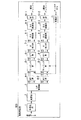•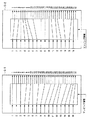•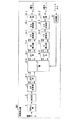•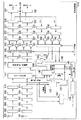•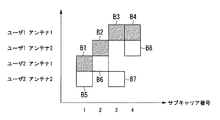•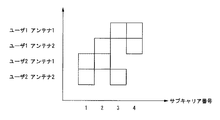•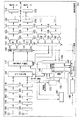•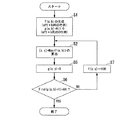•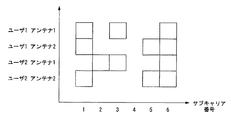•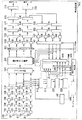•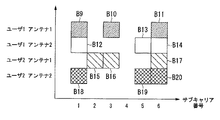•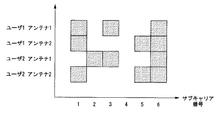•••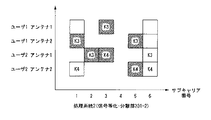•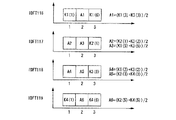••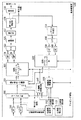•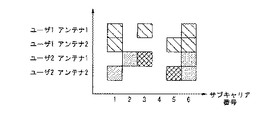•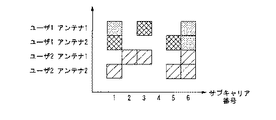•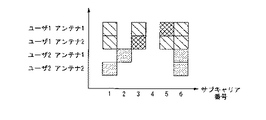•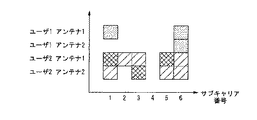••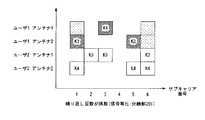••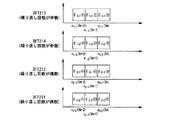•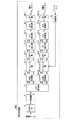•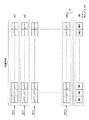•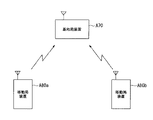•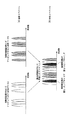••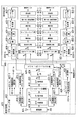•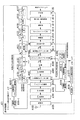•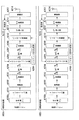•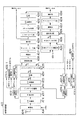•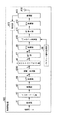•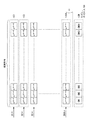•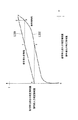•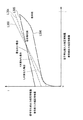••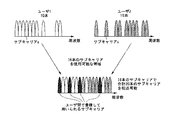••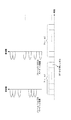•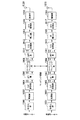••••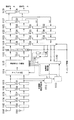•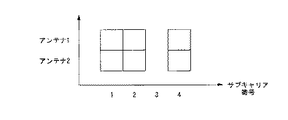•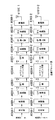•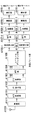•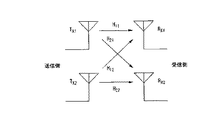## Classifications

• HELECTRICITY
• H04ELECTRIC COMMUNICATION TECHNIQUE
• H04WWIRELESS COMMUNICATION NETWORKS
• H04W72/00Local resource management, e.g. wireless traffic scheduling or selection or allocation of wireless resources
• H04W72/04Wireless resource allocation
• H04W72/044Wireless resource allocation where an allocation plan is defined based on the type of the allocated resource
• H04W72/0453Wireless resource allocation where an allocation plan is defined based on the type of the allocated resource the resource being a frequency, carrier or frequency band
• HELECTRICITY
• H04ELECTRIC COMMUNICATION TECHNIQUE
• H04LTRANSMISSION OF DIGITAL INFORMATION, e.g. TELEGRAPHIC COMMUNICATION
• H04L25/00Baseband systems
• H04L25/02Details ; Arrangements for supplying electrical power along data transmission lines
• H04L25/0202Channel estimation
• H04L25/0204Channel estimation of multiple channels
• HELECTRICITY
• H04ELECTRIC COMMUNICATION TECHNIQUE
• H04LTRANSMISSION OF DIGITAL INFORMATION, e.g. TELEGRAPHIC COMMUNICATION
• H04L25/00Baseband systems
• H04L25/02Details ; Arrangements for supplying electrical power along data transmission lines
• H04L25/0202Channel estimation
• H04L25/024Channel estimation channel estimation algorithms
• H04L25/0242Channel estimation channel estimation algorithms using matrix methods
• HELECTRICITY
• H04ELECTRIC COMMUNICATION TECHNIQUE
• H04LTRANSMISSION OF DIGITAL INFORMATION, e.g. TELEGRAPHIC COMMUNICATION
• H04L25/00Baseband systems
• H04L25/02Details ; Arrangements for supplying electrical power along data transmission lines
• H04L25/03Shaping networks in transmitter or receiver, e.g. adaptive shaping networks ; Receiver end arrangements for processing baseband signals
• H04L25/03006Arrangements for removing intersymbol interference
• H04L25/03159Arrangements for removing intersymbol interference operating in the frequency domain
• HELECTRICITY
• H04ELECTRIC COMMUNICATION TECHNIQUE
• H04LTRANSMISSION OF DIGITAL INFORMATION, e.g. TELEGRAPHIC COMMUNICATION
• H04L5/00Arrangements affording multiple use of the transmission path
• H04L5/0001Arrangements for dividing the transmission path
• H04L5/0003Two-dimensional division
• H04L5/0005Time-frequency
• H04L5/0007Time-frequency the frequencies being orthogonal, e.g. OFDM(A), DMT
• HELECTRICITY
• H04ELECTRIC COMMUNICATION TECHNIQUE
• H04LTRANSMISSION OF DIGITAL INFORMATION, e.g. TELEGRAPHIC COMMUNICATION
• H04L5/00Arrangements affording multiple use of the transmission path
• H04L5/0001Arrangements for dividing the transmission path
• H04L5/0026Division using four or more dimensions
• HELECTRICITY
• H04ELECTRIC COMMUNICATION TECHNIQUE
• H04LTRANSMISSION OF DIGITAL INFORMATION, e.g. TELEGRAPHIC COMMUNICATION
• H04L5/00Arrangements affording multiple use of the transmission path
• H04L5/003Arrangements for allocating sub-channels of the transmission path
• H04L5/0037Inter-user or inter-terminal allocation
• HELECTRICITY
• H04ELECTRIC COMMUNICATION TECHNIQUE
• H04LTRANSMISSION OF DIGITAL INFORMATION, e.g. TELEGRAPHIC COMMUNICATION
• H04L5/00Arrangements affording multiple use of the transmission path
• H04L5/003Arrangements for allocating sub-channels of the transmission path
• H04L5/0044Arrangements for allocating sub-channels of the transmission path allocation of payload
• HELECTRICITY
• H04ELECTRIC COMMUNICATION TECHNIQUE
• H04WWIRELESS COMMUNICATION NETWORKS
• H04W72/00Local resource management, e.g. wireless traffic scheduling or selection or allocation of wireless resources
• H04W72/04Wireless resource allocation
• H04W72/044Wireless resource allocation where an allocation plan is defined based on the type of the allocated resource
• H04W72/0446Wireless resource allocation where an allocation plan is defined based on the type of the allocated resource the resource being a slot, sub-slot or frame
• HELECTRICITY
• H04ELECTRIC COMMUNICATION TECHNIQUE
• H04LTRANSMISSION OF DIGITAL INFORMATION, e.g. TELEGRAPHIC COMMUNICATION
• H04L25/00Baseband systems
• H04L25/02Details ; Arrangements for supplying electrical power along data transmission lines
• H04L25/03Shaping networks in transmitter or receiver, e.g. adaptive shaping networks ; Receiver end arrangements for processing baseband signals
• H04L25/03006Arrangements for removing intersymbol interference
• H04L2025/0335Arrangements for removing intersymbol interference characterised by the type of transmission
• H04L2025/03375Passband transmission
• H04L2025/03414Multicarrier
• HELECTRICITY
• H04ELECTRIC COMMUNICATION TECHNIQUE
• H04LTRANSMISSION OF DIGITAL INFORMATION, e.g. TELEGRAPHIC COMMUNICATION
• H04L25/00Baseband systems
• H04L25/02Details ; Arrangements for supplying electrical power along data transmission lines
• H04L25/03Shaping networks in transmitter or receiver, e.g. adaptive shaping networks ; Receiver end arrangements for processing baseband signals
• H04L25/03006Arrangements for removing intersymbol interference
• H04L2025/0335Arrangements for removing intersymbol interference characterised by the type of transmission
• H04L2025/03426Arrangements for removing intersymbol interference characterised by the type of transmission transmission using multiple-input and multiple-output channels
• HELECTRICITY
• H04ELECTRIC COMMUNICATION TECHNIQUE
• H04LTRANSMISSION OF DIGITAL INFORMATION, e.g. TELEGRAPHIC COMMUNICATION
• H04L25/00Baseband systems
• H04L25/02Details ; Arrangements for supplying electrical power along data transmission lines
• H04L25/0202Channel estimation
• H04L25/022Channel estimation of frequency response
• HELECTRICITY
• H04ELECTRIC COMMUNICATION TECHNIQUE
• H04LTRANSMISSION OF DIGITAL INFORMATION, e.g. TELEGRAPHIC COMMUNICATION
• H04L25/00Baseband systems
• H04L25/02Details ; Arrangements for supplying electrical power along data transmission lines
• H04L25/03Shaping networks in transmitter or receiver, e.g. adaptive shaping networks ; Receiver end arrangements for processing baseband signals
• H04L25/03006Arrangements for removing intersymbol interference
• H04L25/03171Arrangements involving maximum a posteriori probability [MAP] detection
• HELECTRICITY
• H04ELECTRIC COMMUNICATION TECHNIQUE
• H04LTRANSMISSION OF DIGITAL INFORMATION, e.g. TELEGRAPHIC COMMUNICATION
• H04L25/00Baseband systems
• H04L25/02Details ; Arrangements for supplying electrical power along data transmission lines
• H04L25/03Shaping networks in transmitter or receiver, e.g. adaptive shaping networks ; Receiver end arrangements for processing baseband signals
• H04L25/03006Arrangements for removing intersymbol interference
• H04L25/03178Arrangements involving sequence estimation techniques
• H04L25/03312Arrangements specific to the provision of output signals
• H04L25/03318Provision of soft decisions
• HELECTRICITY
• H04ELECTRIC COMMUNICATION TECHNIQUE
• H04LTRANSMISSION OF DIGITAL INFORMATION, e.g. TELEGRAPHIC COMMUNICATION
• H04L27/00Modulated-carrier systems
• H04L27/26Systems using multi-frequency codes
• H04L27/2601Multicarrier modulation systems
• H04L27/2602Signal structure
• H04L27/2605Symbol extensions
• H04L27/2607Cyclic extensions
• HELECTRICITY
• H04ELECTRIC COMMUNICATION TECHNIQUE
• H04LTRANSMISSION OF DIGITAL INFORMATION, e.g. TELEGRAPHIC COMMUNICATION
• H04L27/00Modulated-carrier systems
• H04L27/26Systems using multi-frequency codes
• H04L27/2601Multicarrier modulation systems
• H04L27/2614Peak power aspects
• H04L27/2615Reduction thereof using coding

## Description

The present invention relates to a communication device and a communication method.
This application claims priority on August 13, 2007 based on Japanese Patent Application No. 2007-210936 and Japanese Patent Application No. 2007-210937 for which it applied to Japan, and uses the content here.

In recent years, research on next-generation mobile communication systems has been actively conducted, and as a method for improving the frequency utilization efficiency of the system, each cell uses the same frequency band, so that each cell is allocated to the system. A one-frequency repetitive cellular system that can be used as a whole has been proposed.
In the downlink (communication from the base station apparatus to the mobile station apparatus), the OFDMA (Orthogonal Frequency Division Multiple Access) method is the most promising candidate. In the OFDMA scheme, communication is performed by applying different modulation schemes to information data according to reception conditions such as 64-QAM (64-ary quadrature amplitude modulation) and BPSK (Binary Phase Shift Keying). This is a system that performs communication by flexibly allocating radio resources composed of a time axis and a frequency axis to a plurality of mobile terminal apparatuses using an OFDM signal.

In this case, since the OFDM signal is used, the PAPR (Peak to Average Power Ratio) may be very high, and the high peak power is a downlink with a relatively large margin for the transmission power amplification function. However, in the uplink (communication from the mobile station apparatus to the base station apparatus) where the transmission power amplification function is not sufficient, it becomes a fatal problem.
Therefore, in the uplink (communication from the mobile station apparatus to the base station apparatus), a communication system based on a single carrier system with a low peak-to-average power ratio PAPR is desirable.

However, when the single carrier method is used, there is a problem that flexible resource allocation using the time axis and the frequency axis as in the OFDM method cannot be performed. SC-ASA (Single Carrier-Adaptive Spectrum Allocation: also referred to as DFT-S-OFDM (Discrete Fourier Transform-Spread OFDM)) has been proposed as a communication method for solving this problem. (For example, Non-Patent Document 1).
Since such a communication method uses the same method as the single carrier communication method, the peak-to-average power ratio PAPR becomes low. Moreover, it becomes possible to process data without inter-block interference by inserting a cyclic prefix as in an OFDM signal (in this specification, an interval for inserting a cyclic prefix, that is, a data processing unit for performing DFT) Are referred to as DFT-S-OFDM symbols). Further, since the frequency waveform is once generated by DFT, there is an advantage that resource control in units of subcarriers can be easily performed.

FIG. 40 shows the configuration of a transmission apparatus when MIMO (Multi-Input Multi-Output) transmission is performed using this SC-ASA system. However, FIG. 40 may be viewed as one transmission device including a plurality of transmission systems, or may be viewed as different transmission devices. This point will be described next. In FIG. 41A, one base station performs radio communication with two mobile stations. Each base station and mobile station each have two antennas. 40 is a single-user MIMO transmission in FIG. 41C when viewed as a single transmission device having a plurality of transmission systems, and a multi-user MIMO transmission in FIG. 41B when viewed as different transmission devices. Become. However, the subcarriers to be used are represented by white blocks, and the subcarriers with numbers without white blocks are not selected in the SC-ASA system.

In each transmission system of FIG. 40, transmission data 1 and 2 are encoded by encoding units 1000 and 1001, and modulated by modulation units 1002 and 1003. The modulated signals are parallelized by S / P (serial / parallel) converters 1004 and 1005 and converted into signals on the frequency axis by DFT units 1006 and 1007. In the two spectrum mapping units 1008 and 1009, as shown in FIGS. 41B and 41C, the signals of transmission data 1 and transmission data 2 are mapped so as to use subcarriers of the same frequency. When the SC-ASA method is used, subcarriers with high reception SNR and SINR are used. However, since the transmission is MIMO transmission, signals transmitted from the two transmission systems interfere with each other on the reception side. End up. Therefore, in order to consider the degree of interference with each other, a subcarrier having a good result considering all the propagation paths of the two transmission systems and the two reception systems must be selected in common by the transmission antenna (user). .

Next, the mapped transmission signal on the frequency axis is converted into a time-axis signal in IDFT sections 1010 and 1011, and the signals are serialized in P / S (parallel / serial) conversion sections 1012 and 1013. Thereafter, cyclic prefix CP insertion sections 1014 and 1015 insert cyclic prefixes, and D / A conversion sections 1016 and 1017 convert them into analog signals. Finally, the radio units 1018 and 1019 up-convert to radio frequencies and transmit from the respective transmission antennas 1020 and 1021.

FIG. 42 is a schematic block diagram showing a configuration of a receiving apparatus that receives a signal transmitted in this way through MIMO transmission. However, the receiving apparatus shown in FIG. 42 has a canceller, and good characteristics can be obtained by receiving with the receiving apparatus having such a configuration. 42 includes antenna units 1100 and 1101, RF units 1102 and 1103, A / D conversion units 1104 and 1105, CP removal units 1106 and 1107, S / P conversion units 1108, 1109, 1133 and 1134, and a DFT unit. 1110, 1111, 1116, 1117, 1135, 1136, propagation path estimation units 1112, 1113, cancellation unit 1114, signal equalization / separation unit 1115, spectrum demapping unit 1118, IDFT units 1119, 1120, 1138, 1139, P / From the S converters 1121 and 1122, the demodulator 1123 and 1124, the decoders 1125 and 1126, the iterative controllers 1127 and 1128, the determination units 1129 and 1130, the replica generators 1131 and 1132, the spectrum mapping unit 1137, and the propagation path multiplier 1140 Composed

The signals transmitted from the transmission device shown in FIG. 40 are first received by the antenna units 1100 and 1101, respectively, in the reception device, down-converted from the radio frequency by the RF units 1102 and 1103, and then A / D conversion units 1104 and 1105. After being converted into a digital signal, the cyclic prefix CP (GI) added on the transmission side is removed by the CP removal sections 1106 and 1107. Next, the S / P converters 1108 and 1109 convert the signals into parallel signals, and the DFT units 1110 and 1111 perform DFT processing to convert the signals into frequency domain signals. Of the signals converted into the frequency domain in this way, the propagation path estimation unit 1112, 1113 performs propagation path estimation between each transmission antenna and the reception antenna using a known signal added on the transmission side for propagation path estimation. Is called. In this example, propagation path estimation values for the number of transmission antennas × number of reception antennas = 4 paths are calculated by the number of subcarriers.

The data signal that has been subjected to DFT processing and converted into a frequency domain signal is input to the cancel unit 1114. The cancel unit 1114 performs subtraction between the reception signal and the replica of the reception signal generated based on the reliability of the demodulated data, and when a complete replica (transmission signal) can be generated, this output includes only the noise component. It becomes. This calculation is performed when R is a reception data vector received by two antennas, Ξ is a propagation path matrix, and S ′ is a replica of a transmission data vector (generated in a replica generation unit to a spectrum mapping unit described later). It is expressed by the formula (100).

However, Q is a vector representing the output (residual after cancellation) of the cancel unit 1114 at the second and subsequent iterations, and R, Ξ, and S ′ are expressed by the following equations (101) to (103). The In these equations, the numbers in parentheses are subcarrier numbers, and the subscripts represent transmission and reception antenna numbers. Further, two subscripts of “表” represent a combination of a reception antenna and a transmission antenna. For example, “Ξ” 21 represents a propagation path from the transmission antenna 1 to the reception antenna 2. These equations can be used for either single user-MIMO or multi-user-MIMO.

Here, the reason for canceling all replicas (ΞS ′) including the desired signal to be extracted is that the signal equalization / separation unit 1115 described later involves an inverse matrix operation, so that only the desired signal remains and is canceled and equalized. Is repeated, it is necessary to perform inverse matrix operation as many times as desired signals included in the block, but by inputting the canceled residual Q, the residual can be handled in common in the block. Since all the weights can be calculated if the inverse matrix operation is performed once, the amount of calculation associated with the inverse matrix operation is reduced by inputting a replica separately and reconfiguring it. However, since a replica of the received signal cannot be generated at the first time, the received data vector (R) passes through the cancel unit 1115 as it is.

The signal that has passed through the cancel unit 1114 is input to the signal equalization / separation unit 1115 and equalized using the frequency domain signal. When iterative processing is performed, the signal equalization / separation unit 1115 adds a replica of the received signal for each data vector transmitted from the antenna 1 and the antenna 2 in FIG. 43 to the output (Q) of the canceller unit. On the other hand, equalization processing based on the MMSE standard is performed using equation (104). FIG. 43 is a diagram illustrating transmission of signals of subcarriers 1, 2, and 4 from antennas 1 and 2, respectively, as an example of subcarrier selection.

However, Tn (n = 1, 2 in the above example) represents a transmission antenna, γ Tn and δ Tn are real numbers expressed by calculating tap coefficients, and Ψ Tn is the size of the DFT block length also expressed by calculating tap coefficients. Is a complex square matrix. Further, s ′ Tn represents a replica of the signal transmitted from the antenna Tn, and Q represents a result (residual) obtained by subtracting the replica of all received signals from the received signal. However, since a replica of the received signal cannot be generated in the first process (s ′ Tn is a zero vector), the equalization process is performed on the signal R that has passed through the cancel unit 1114 without any subtraction. When calculating Ψ Tn and the like of the equation (104), the propagation channel matrices Ｔ T1 and Ξ T2 for the transmission data vectors 1 and 2 are used in addition to the propagation channel matrix of the equation (102). Here, Ξ T1 is a propagation path matrix for each transmission antenna, and Ξ T2 is used when equalizing the transmission data vector 2 and Ξ T2 is equalizing the transmission data vector 2, respectively.

By the equalization calculation using the equation (104), the signal equalization / separation unit 1115 outputs a signal after equalization in the time domain for each transmission data (for example, described in Non-Patent Document 3).
Signals equalized for each signal transmitted from each transmission antenna are then input to DFT sections 1116 and 1117, converted into frequency domain signals, and then input to spectrum demapping section 1118. The spectrum demapping unit 1118 performs demapping common to the spectrums transmitted from the antennas 1 and 2 based on the used spectrum mapping information. In the IDFT units 1119 and 1120, each demapped signal sequence is converted into a time domain signal, and then converted into a serial signal in the P / S conversion units 1121 and 1122, and demodulation and decoding processes are performed.

Demodulating sections 1123 and 1124 calculate an LLR (Log Likelihood Ratio) representing the reliability of error-corrected received data. Further, the decoding units 1125 and 1126 perform error correction code decoding processing on the LLR to update the LLR. The repetition control units 1127 and 1128 to which the LLR is input determine whether or not a predetermined number of repetition processes have been performed. If the predetermined number of repetition processes has been performed, the LLR determination unit is determined. 1129 and 1130. Conversely, if the number of repetition processes is less than the predetermined number, the LLR is output to the replica generation units 1131 and 1132, and the process proceeds to reception signal replica generation processing. If it is assumed that a CRC (Cyclic Redundancy Check) code is used, iterative processing may be terminated when no error is detected.
In replica generation sections 1131 and 1132, signal replicas (transmission signal replicas) corresponding to the LLRs of the respective bits are respectively generated, transmitted through S / P conversion sections 1133 and 1134, and transmitted from respective transmission antennas in DFT sections 1135 and 1136. Each of the converted signals is converted to a frequency domain replica.

The frequency domain signal replica generated in this way is then mapped in the spectrum mapping unit 1137 in the same manner as on the transmission side, based on the mapping information notified from the use spectrum determining unit (not shown). The spectrum-mapped replica S ′ is input to the propagation path multiplying unit 1140 and also input to the signal equalizing / separating unit 1115 via the IDFT units 1138 and 1139. The signal equalization / separation unit 1115 to which the replica S ′ after the spectrum mapping is input reconstructs the reception signals of the transmission data vectors 1 and 2 using the replica as described above, and transmits each of the transmission signals. Used for data vector equalization. In addition, propagation path multiplying section 1140 multiplies the replica after spectrum mapping by a propagation path matrix (Ξ in equation (102)) in order to generate a replica of the received signal used for subtraction with the received signal by cancellation section 1114. . The replica (レ プ リ カ S ′) of the received signal output from the propagation path multiplying unit 1140 is input to the canceling unit 1114, and the subtraction of the equation (100) described above is performed.

In the receiving apparatus shown in FIG. 42, the reliability of decoded bits is gradually improved by repeating the processes such as replica cancellation, equalization, spatial / spectrum demapping, decoding, and replica generation as described above. Go. Then, after performing a predetermined number of repetitions, the determination units 207 and 208 perform a hard bit determination, and the transmission data is reproduced as decoded data.

In addition, as a system for multiplexing transmission data from a plurality of transmission stations using the SC-ASA system, the number of points of inverse discrete Fourier transform IDFT (Inverse Discrete Fourier Transform) from the number of points of discrete Fourier transform DFT at the transmission station An FDMA (Frequency Division Multiple Access) type in which subcarriers with 0 input are used by other transmitting stations is proposed (for example, Non-Patent Document 3). .

44A and 44B are schematic block diagrams illustrating configurations of a transmitting station apparatus and a receiving station apparatus when user multiplexing is performed by applying the SC-ASA communication method in two conventional transmitting stations. 44A, two sets of transmission data 1 and transmission data 2 are encoded by encoding section A1000-1 and encoding section A1000-2, respectively, and the encoded transmission data is modulated by modulation section A1001-. 1. Modulation is performed by the modulation unit A1001-2. The modulated signals modulated by the modulation unit A1001-1 and the modulation unit A1001-2 are parallelized by the S / P (serial / parallel) conversion unit A1002-1 and the S / P conversion unit A1002-2, and then the DFT unit A1003-. 1. It is converted into a signal on the frequency axis by the DFT unit A1003-2. Thereafter, the signals on the frequency axis are mapped to subcarriers by the spectrum mapping unit A1004-1 and the spectrum mapping unit A1004-2 so that the transmission data 1 and transmission data 2 signals do not use subcarriers having the same frequency. Is done. At this time, the mapping to the subcarrier is a subcarrier of a frequency having a good reception SNR (Signal to Noise Ratio) or SNIR (Signal to Noise Interference Ratio), and others. Assigned to frequencies not used by other users.

Next, the mapped transmission signal on the frequency axis is converted into a time axis signal by IDFT unit A1005-1 and IDFT unit A1005-2, and P / S (parallel / serial) conversion unit A1006-1, P / S. The time axis signal is serialized by the conversion unit A1006-2. Thereafter, the serialized signal is inserted with a cyclic prefix by a CP (cyclic prefix) insertion unit A1007-1 and a CP insertion unit A1007-2, and a D / A conversion unit A1008-1 and a D / A conversion unit A1008. -2 is converted to an analog signal. Finally, the analog signal is up-converted to a radio frequency by the radio unit A1009-1 and the radio unit A100902, and transmitted from the transmission antenna 1010-1 and the transmission antenna 1010-2 of each transmission station.

In the receiving station apparatus of FIG. 44B, a reception signal obtained by combining two signals transmitted simultaneously is received by reception antenna 1100, and this reception signal is down-converted by radio section A1111. The down-converted received signal is converted into a digital signal by the A / D converter A1101. Next, the cyclic prefix is removed from the digital signal by a CP (cyclic prefix) removal unit A1102, and the digital signal from which the cyclic prefix is removed is parallelized by the S / P conversion unit A1103. The parallelized digital signal is converted into a frequency axis signal by the DFT unit A1104, and each subcarrier of the frequency axis signal is returned to the original arrangement by the spectrum demapping unit A1105. The signal from the transmitting station is separated. Thereafter, each transmission data is independently equalized by the signal equalization unit A1106-1 and the signal equalization unit A1106-2, and is converted into a time-axis signal by the IDFT unit A1107-1 and the IDFT unit A1107-2. Thereafter, the signals are serialized by the P / S converter A1108-1 and the P / S converter A1108-2, demodulated by the demodulator A1109-1 and the demodulator A1109-2, and decoded by the decoder A1110-1 and decoder A1110-2. Decoded data 1 and decoded data 2 of the signal transmitted from each transmitting station are obtained.

Here, as an equalization method of the signal equalization unit A1106, a method of a minimum mean square error (MMSE) standard or the like is used. In general, the equalization processing based on the MMSE norm calculates a tap that minimizes the evaluation function J expressed by Expression (107).

In Equation (107), where E [x] is an average value of x, W is a complex tap matrix in which each column vector is an optimal tap vector in each symbol in the DFT-S-OFDM symbol, r is a complex time base received signal vector, and s is a time base transmit signal vector. The right shoulder A H represents the Hermitian transpose of the matrix A. At this time, the optimum tap coefficient W is called a Weiner solution, and is expressed by Expression (108).

In Expression (108), H represents a channel matrix in the time domain, σ 2 represents noise variance, and I represents a unit matrix. Here, especially when signal processing is performed in the frequency domain, a matrix in which the frequency response obtained by Fourier transform from the impulse response of the propagation path is arranged in a diagonal component can be handled as a propagation path matrix. Assuming that 周波 数 is used, when a frequency domain received signal is used, the tap coefficient in equation (108) can be transformed as in the following equation (109).

In Expression (109), F represents a matrix that performs DFT processing, and F H represents a matrix that performs inverse DFT processing. When this tap matrix is multiplied with the received signal r in the time domain, the equalized received signal z is expressed by Equation (110).

However, R = Fr, that is, the received signal r converted into a signal on the frequency axis by DFT processing. From equation (110), when a normal received signal is input and equalized in the frequency domain, the received signal is transformed by DFT, and Hermitian transposition of a matrix obtained by removing F H and F on both sides of equation (109) is performed. The operation of multiplying and returning to the time signal by IDFT is performed. Therefore, a normal MMSE filter receives the frequency axis received signal and the frequency response of the propagation path, and outputs the frequency axis equalized signal.

On the other hand, when the configuration of the receiving station apparatus performs iterative processing using a canceller such as SC / MMSE (Soft Canceller followed by MMSE), a signal generated according to the reliability of the bits obtained from the decoding unit In order to improve the accuracy of the signal input to the equalization unit by canceling the interference wave from the received signal using the replica, the input signal of the equalization unit differs for each repetition. For this reason, the term corresponding to the received signal r of the evaluation function represented by the equation (107) is a signal other than the desired signal, and the equalized signal is represented by the following equation (111).

In equation (111), R rest is the remaining amount that cannot be canceled by subtracting the received signal replica generated by multiplying the time-axis signal replica by the propagation path characteristic from the actual received signal on the time axis. The difference, s rep (k), is the transmitted signal replica in the k th sample. Γ and δ are real numbers expressed by the tap coefficient calculation, and Ψ is a complex square matrix having the size of the DFT-S-OFDM symbol length similarly expressed by the tap coefficient calculation. These are all channel characteristics in the frequency domain. And frequency axis signal replicas (for example, described in Non-Patent Document 2). Further, in the case of the first processing in the formula (111), no replica is input (s rep (k) = 0), so in this case, the optimum tap of the formula (107) is obtained, and the formula (111) is the formula (109). Matches.

Therefore, in the equalization by SC / MMSE, a residual on the frequency axis is input as an input signal, a replica of the time axis signal and a propagation path characteristic of the frequency axis are input, and a time axis signal is output. As shown in Expression (111), the process of canceling other than the desired component is performed by calculating the residual R rest and then reconfiguring the desired component using the transmission signal replica and the propagation path characteristics. Not only can be expressed uniquely in the S-OFDM symbol, but also the residual R rest can be the same in the DFT-S-OFDM symbol, so that the amount of computation involving inverse matrix computation can be reduced.

Study on broadband single-carrier transmission system using dynamic spectrum control, RCS2006, January 2007 M. Tuchler and J. Hagenauer, "Linear time and frequency domain turbo equalization," Proc. VTC, pp. 2773-2777, Rhodes, Greece, Oct. 2001. Study on co-channel interference countermeasure using dynamic spectrum control in multi-user MIMO system, IEICE 2006 General Conference, March 2007

In conventional multi-user MIMO and single-user MIMO, as described above, transmission is performed from a plurality of antennas at a selected frequency. In this case, considering all the propagation paths between a plurality of transmission systems and a plurality of reception systems, control using subcarriers having relatively good propagation paths (or assigning users) was performed. . Specifically, in the case of 2 × 2 MIMO transmission (which may be either multi-user MIMO or single-user MIMO) having transmission antennas Tx1 and Tx2 and reception antennas Rx1 and Rx2 as shown in FIG. 45, four propagation paths Considering all of H11, H21, H12, and H22, subcarriers having relatively good propagation paths are selected by both of the transmission antennas Tx1 and Tx2, and different data are transmitted simultaneously from Tx1 and Tx2. This is because when MIMO transmission is performed, signals transmitted from a plurality of transmission antennas interfere with each other, and as a result of considering all of them, it is necessary to select the best subcarrier.

However, when the SC-ASA scheme is applied to MIMO transmission, such control does not result in selection that is considered to provide the highest selection diversity effect, and may not provide good frequency utilization efficiency. There is. In other words, the SC-ASA scheme is originally a scheme in which a high selection diversity effect is obtained by selecting and transmitting an optimal subcarrier for each transmission apparatus (transmission antenna), and frequency utilization efficiency is increased. When applied to the above-described conventional MIMO transmission (multi-user MIMO or single-user MIMO), the selection of the optimal subcarrier is not independent for each transmission antenna, so that good frequency utilization efficiency may not be obtained. There is a problem that there is.

Conventionally, when transmitting from a plurality of transmission apparatuses using the SC-ASA scheme, subcarriers are allocated so as to avoid frequencies used by other transmission apparatuses. For this reason, if a transmission apparatus is already assigned to another transmission apparatus even though it is a subcarrier having good reception SNR and SINR, the above-described transmission apparatus cannot be used. Even if there is a subcarrier having a good reception SNR or SINR within the transmittable band, when other devices are communicating, the subcarrier cannot be used. Therefore, there is a problem that good frequency utilization efficiency cannot be obtained.

The problem to be solved is that good frequency utilization efficiency can be obtained even when the SC-ASA scheme is applied to transmission from a plurality of transmission apparatuses or MIMO transmission.

(1) The present invention has been made to solve the above-described problem, and a communication apparatus according to an embodiment of the present invention transmits a first subcarrier composed of a plurality of subcarriers. Transmission using one received signal and a second subcarrier consisting of one or more subcarriers overlapping with the first subcarrier and one or more subcarriers not overlapping with the first subcarrier in a time frame And a reception unit that receives a reception signal including at least a second reception signal to be transmitted, and the reception signal is transmitted using the subcarrier in which the first subcarrier and the second subcarrier overlap A demodulator that removes the second received signal and demodulates information about bits included in the first received signal.

(2) The reception unit of the communication device described above receives the first reception signal from the first communication device and receives the second reception signal from the second communication device.

(3) The reception unit of the above-described communication device is characterized in that it includes a smaller number of antennas than the number of reception signals received in the time frame.

(4) The receiving unit of the above-described communication apparatus is configured by one receiving antenna.

(5) The demodulator of the above-described communication apparatus demodulates information about the bit from the first received signal using iterative processing.

(6) In the above base station apparatus, the number of overlapping subcarriers is set for each time frame.

(7) A communication method according to another embodiment of the present invention includes a first received signal transmitted using a first subcarrier composed of a plurality of subcarriers, and the first subcarrier in a time frame. Receiving a reception signal including at least a second reception signal transmitted using a second subcarrier including one or more subcarriers overlapping with the first subcarrier and one or more subcarriers not overlapping with the first subcarrier. And, from the received signal, removing the second received signal transmitted using the one or more subcarriers in which the first subcarrier and the second subcarrier overlap, and And at least a step of demodulating information about bits included in the received signal.

According to the present invention, even when the SC-ASA scheme is applied to transmission from a plurality of transmission apparatuses or MIMO transmission, it is possible to select an appropriate subcarrier and obtain good frequency utilization efficiency.

[First Embodiment]
In the first embodiment, a method of determining a subcarrier to be used by data transmitted from each antenna based on a channel state for each subcarrier of each antenna will be described. The radio communication system according to the present embodiment includes a plurality of mobile station apparatuses and base station apparatuses. The base station device is a receiving device in the present embodiment, and the mobile station device is a transmitting device in the present embodiment. In addition, the number of mobile station apparatuses that are simultaneously connected to the base station apparatus is 2, and the number of transmission antennas provided in each mobile station apparatus is 2, and signals are transmitted from a total of 4 transmission antennas. The transmission method of the mobile station apparatus is DFT-S-OFDM, the total number of subcarriers is 32, and the number of subcarriers used by each antenna of each mobile station apparatus is 16. In this embodiment, the number of reception antennas of the base station apparatus is set to 2, and in order to separate the reception signals from these two antennas for each transmission antenna, a total of four transmissions are performed simultaneously on one subcarrier. Any two of the antennas.

FIG. 1 is a control flow regarding a method of selecting a subcarrier used for data transmission in the present embodiment. For convenience, the antenna numbers of the transmission antennas of the user 1 are “1” and “2”, and the antenna numbers of the transmission antennas of the user 2 are “3” and “4”. In FIG. 1, step S1 is a process for generating two functions f (a, b) and g (a, b). Each is a matrix of a rows and b columns. a is represented by the number of users × the number of transmission antennas, and in this embodiment, 2 × 2 = 4. Further, b is the number of subcarriers, and is 32 in this embodiment. Here, as the value of the function f, the channel capacity C m (k) of each subcarrier transmitted from each transmission antenna represented by the following equation (1), or a constant term is omitted from the equation (1). For example, a value corresponding to the channel capacity that simplifies the calculation within a range where the magnitude relationship does not change is used. Here, m is a transmission antenna number, k is a subcarrier number, NT is the number of transmission antennas, Es is transmission energy of one user, and ξ m (k) is a kth subcarrier transmitted from the mth transmission antenna. Σm (k) represents the interference noise power in the kth subcarrier transmitted from the mth transmission antenna. Here, Σm (k) is expressed by Equation (1 ′), where η (k) is the interference noise vector at each receiving antenna.

However, in Formula (1 ′), E [x] represents the set average of x, and ‖x‖ represents the norm of the vector x.
This equation (1) is an equation representing the channel capacity for each subcarrier transmitted from each transmitting antenna at the time of SIMO (Single Input Multi Output) transmission, and this can be used as a selection criterion for the used subcarrier. Is a case where all components that interfere with each other between signals transmitted from the respective transmitting antennas can be removed by processing on the receiving side, which will be described later. In such a case, it is called a complete convergence state in the reception process, and the reception characteristic at the time of SIMO transmission is obtained. Therefore, by selecting transmission subcarriers independently at each transmission antenna based on the expression (1), it is more flexible than the conventional selection methods, and a higher selection diversity effect can be obtained.

In addition, all values of the function g are initialized to zero. By performing the processing shown in the flow of FIG. 1, this function g becomes a function indicating the presence / absence of signal transmission in a combination of an input antenna number and a subcarrier number. For example, when g (2, 5) = 1. Means that the signal is transmitted on the 5th subcarrier of the 2nd antenna. Next, step S2 is a step of extracting the element number of the matrix having the maximum value in the function f. That is, x and y of x rows and y columns in which elements of maximum values are arranged are calculated. Next, step S4 is a step of determining whether or not the number of transmission antennas already assigned to the y-th column of the function g is one or less. In other words, it is a step of determining whether or not the number of transmission antennas for transmitting signals on the y-th subcarrier is one or less. The number is calculated and the determination is made.

This one or less constraint in step S4 depends on the assumption that the maximum number of transmission antennas allocated to the same subcarrier is 2, which is the precondition previously. In step S4, it is determined that the number is 1 or less. If allocation is still possible (S4-Yes), g (x, y) = 1 is set in step S5 and the allocation is reflected in the function g. In step S6, the number of 1s of the function g is counted and compared with 64. Since this value is incremented by 1, when it becomes 64, it means that all assignments have been completed. However, 64 = the number of used subcarriers of each antenna (16) × the total number of antennas of the mobile station apparatuses connected simultaneously (4). If two antennas are already assigned in step S4, and if it is determined in step S6 that assignment is still necessary, the value of the function f relating to the elements x and y currently selected in step S7 is- 100. This value of -100 is not meaningful, but is changed to a small value so that the element is not selected again in step S2.

The results selected in this way are shown in FIG. In FIG. 2, the vertical axis represents the antenna number for each mobile station apparatus, and the horizontal axis represents the subcarrier number. A subcarrier surrounded by a square means a subcarrier used for signal transmission. In the example shown in FIG. 2, the subcarriers of No. 30 and No. 32 are in the same state as single user-MIMO in which signals are multiplexed by one mobile station apparatus. That is, the subcarrier 30 is used by the antennas 1 and 2 of the user 1, and the subcarrier 32 is used by the antennas 1 and 2 of the user 2. The subcarriers No. 1, 2, 3, and 31 are in the same state as multiuser-MIMO that shares subcarriers used by a plurality of mobile station apparatuses and multiplexes signals. That is, for example, the subcarrier 1 is used by the antenna 1 of the user 1 and the antenna 2 of the user 2. Thus, it can be said that data can be transmitted in a good state by selecting subcarriers that are used independently by each antenna depending only on the state of the transmission path.

Here, antennas that occupy subcarriers are determined only by the state of the transmission path without giving likelihood to all antennas. However, the state of the transmission path is large between the first mobile station apparatus and the second mobile station apparatus. When there is a difference, one mobile station apparatus may not always be able to select a subcarrier in good condition. In order to cope with such a case, a function f is prepared for each mobile station apparatus, and each mobile station apparatus selects a subcarrier in order.

In the above example, the calculation result of the expression (1) is used as the value of the function f. However, unlike this, the transmission path state (gain by propagation path) and SINR for each transmission antenna and each subcarrier are different. (Signal to Interference Noise Ratio: Signal-to-interference noise power ratio (interference here is an unknown interference component coming from an adjacent cell or the like)) or the like may be substituted as the value of the function f. However, since there are two propagation paths for one transmission antenna in this embodiment (because there are two reception antennas of the base station), the transmission path state and SINR observed by the two reception antennas are Each subcarrier is added or averaged and used as each element of the function f. Even when such a simple reference such as the average SINR at each receiving antenna is used, the transmission subcarrier can be selected independently for each transmitting antenna as in the case of using the equation (1).

Next, FIG. 3 shows a configuration of mobile station apparatus 500 for performing transmission on subcarriers independently selected for each transmission antenna of each mobile station apparatus according to the control flow of the present embodiment shown in FIG. It is a schematic block diagram. In FIG. 3, 11 is a receiving unit that receives mapping information indicating designation of subcarriers used for signal transmission for each transmission antenna from the base station apparatus via the antenna, and 1 is an encoding unit that applies error correction code to transmission data. 2 is a modulation unit that performs modulation (hereinafter referred to as “primary modulation”) such as BPSK (Binary Phase Shift Keying) on the output of the encoding unit 1, and 3 is serial input data. This is an S / P (serial / parallel) converter that converts the modulation signal output from the modulation unit 2 into parallel data corresponding to MIMO. Note that the antenna used by the reception unit 11 may also be used as the first antenna or the second antenna.

Since the subsequent circuits assume that there are two transmission antennas, there are two series (x-1, x-2). Reference numeral 4 denotes a DFT unit that performs frequency spreading by performing DFT (Discrete Fourier Transform) conversion on the modulated signal input from the S / P conversion unit 3. 5-1 and 5-2 are mapping units that arrange the signals spread by the DFT units 4-1 and 4-2 on the subcarriers to be used based on the mapping information received by the receiving unit 11 from the base station apparatus. . 5-1 and 5-2 are assigned independent subcarriers, respectively, and the subcarriers are calculated by the function g. In addition, mapping sections 5-1 and 5-2 input “0” to subcarriers to which no data is mapped.

Reference numerals 6-1 and 6-2 denote IDFT sections that perform IDFT (Inverse Discrete Fourier Transform) conversion on the signals mapped to the subcarriers by the mapping sections 5-1 and 5-2. Reference numeral 2 denotes a GI insertion unit that inserts a guard interval into the outputs of the IDFT units 6-1 and 6-2. The GI insertion units 7-1 and 7-2 use a copy of the last part of the input data in the interval of the guard interval, and this is called a cyclic prefix. Here, the reason for using the cyclic prefix will be described. Since the discrete Fourier transform DFT is premised on a waveform having a period that is an integral multiple of one period of the periodic function within the DFT interval, if there is a delayed wave component in the multipath propagation path, the delay in the received signal on the receiving side Since the periodic function of the wave component is lost, the received signal cannot be separated into subcarriers by discrete Fourier transform DFT, and the subcarriers cannot be handled independently.
On the other hand, if a cyclic prefix corresponding to the maximum delay time of the propagation path is inserted in advance on the transmission side, the periodic function can be maintained even for the delayed wave component by deleting it on the reception side. Even if each subcarrier is handled independently, that is, even if it is assigned to an arbitrary frequency, it can be restored on the receiving side.

Reference numerals 8-1 and 8-2 denote P / S (parallel / serial) conversion units for converting the outputs of the GI insertion units 7-1 and 7-2, which are parallel data, into serial data. Is a D / A (digital / analog) converter for converting the outputs of the P / S converters 8-1 and 8-2, which are digital data, into analog data. Reference numerals 10-1 and 10-2 denote RF (Radio Frequency) units each having a function of converting data into a frequency band for transmitting data. An independent antenna is connected to the RF units 10-1 and 10-2, such as a first antenna for the RF unit 10-1 and a second antenna for the RF unit 10-2. In this embodiment, the encoding unit 1 is common to a plurality of transmission signals, but different encoding units may be associated with each signal transmitted from each transmission antenna.

As illustrated in FIG. 3, the mobile station device 500 includes an encoding unit 1, a modulation unit 2, an S / P conversion unit 3, DFT units 4-1 and 4-2, mapping units 5-1 and 5-2, IDFT. Units 6-1 and 6-2, GI insertion units 7-1 and 7-2, P / S conversion units 8-1 and 8-2, D / A conversion units 9-1 and 9-2, and RF unit 10- 1, 10-2 and a receiving unit 11.
With such a configuration, data can be transmitted according to the subcarrier arrangement for each antenna determined by the flow shown in FIG.

Next, in order to explain the state of mapping to subcarriers, the operation of mapping sections 5-1 and 5-2 will be described with reference to user 1 in FIG. However, the subcarriers used by the user 1 and the antenna 1 in FIG. 2 are (subcarrier numbers 1, 3, 5, 8, 10, 11, 14, 17, 20, 22, 24, 25, 28, 30, 31 , 32) and the subcarriers used by user 1 and antenna 2 are (subcarrier numbers 2, 4, 5, 6, 7, 8, 11, 15, 17, 19, 20, 22, 23, 26, 30, 31). Here, the user 1 represents the mobile station device 500 having the mobile station number 1, and the antenna 1 represents the antenna having the first antenna number. The same applies to the user 2 and the antenna 2.

FIG. 4 is a diagram illustrating operations of the mapping units 5-1 and 5-2. The used subcarrier information (mapping information) for each antenna is input from the receiving unit 11 to the mapping units 5-1 and 5-2. In FIG. 4, the left side of the mapping units 5-1 and 5-2 is input (there are 16 inputs since the number of subcarriers used is assumed to be 16), and the right side is output (selection from 32 subcarriers). Therefore, there are 32 outputs). In addition, an output signal without input signal connection is zero. The mapping unit 5-1 illustrated in FIG. 4 assigns the first input signal to the first subcarrier, assigns the second input signal to the third subcarrier, and so on. Since no input signal is assigned to the subcarrier of, 0 is output from the second subcarrier. In FIG. 4, the mapping units 5-1 and 5-2 are shown as configured to select and connect input / output. However, the input signal is input to the memory and called to the output side according to the mapping information. It is good also as a structure.

FIG. 5 is a schematic block diagram showing a configuration of a mobile station apparatus 501 which is a different configuration example of the mobile station apparatus 500. In the figure, blocks having the same functions as those in FIG. In FIG. 5, unlike FIG. 3, the DFT unit has only the DFT unit 4-1 without the DFT unit 4-2, and the mapping unit has 1 of the mapping unit 5-3 without the mapping units 5-1 and 5-2. It is connected. In the mobile station apparatus 501, the mapping unit 5-3 maps the spectrum output from the DFT unit 4-1 for two symbols. Thereby, the transmission signal transmitted from a plurality of transmission antennas is configured. Also, the DFT unit 4-1 in FIG. 5 may have an input / output size twice that of the DFT unit 4-1 in FIG.

In the present embodiment, subcarriers to be used are determined according to the control flow shown in FIG. 1, but this subcarrier may be determined for each frame of the target wireless communication system. By selecting a subcarrier for each frame as described above, it is possible to select a subcarrier corresponding to a change according to the time of the propagation path condition, and the frequency utilization efficiency is further improved.
The base station apparatus will be described in the following embodiments.

As in the flowchart shown in FIG. 1, in this embodiment, transmission subcarriers are selected independently for each antenna to be transmitted, so that the transmission channel having the best condition can be selected from the transmittable propagation paths. . For this reason, high frequency utilization efficiency can be obtained for each mobile station apparatus and the entire system.

[Second Embodiment]
In the second embodiment, a replica of the received signal is generated based on the reliability of demodulated data for a signal in which spatial / spectrum mapping according to the present invention is performed and single user-MIMO and multi-user-MIMO are mixed. After generating and subtracting unnecessary interference (replica) from the received signal, SC / MMSE (Soft Cancer / MMSE) technology is applied to gradually improve the reliability of demodulated data by repeating processes such as equalization and demodulation. The receiving apparatus structure in the case is shown.

Also in this embodiment, as in the first embodiment, the number of mobile station devices that are simultaneously connected to the base station device is 2, and the number of transmission antennas provided in each mobile station device is 2, Signals are transmitted from a total of four transmission antennas. The transmission method of the mobile station apparatus is DFT-S-OFDM, the total number of subcarriers is 32, and the number of subcarriers used by each antenna of each mobile station apparatus is 16. In this embodiment, the number of reception antennas of the base station apparatus is set to 2, and in order to separate the reception signals from these two antennas for each transmission antenna, a total of four transmissions are performed simultaneously on one subcarrier. Any two of the antennas.

FIG. 6 is a schematic block diagram showing a configuration of base station apparatus 510 which is a receiving apparatus in the present embodiment. As shown in FIG. 6, the receiving apparatus in the present embodiment includes antenna units 100 and 101, RF units 102 and 103, A / D conversion units 104 and 105, CP removal units 106 and 107, S / P conversion unit 108, 109, 135, 136, DFT section 110, 111, 116, 117, 137, 138, 139, 140, propagation path estimation section 112, 113, cancellation section 114, signal equalization / separation section 115, space / spectrum demapping section 118, IDFT units 119, 120, 121, 122, 142, 143, P / S conversion units 123, 124, demodulation units 125, 126, decoding units 127, 128, repetition control units 129, 130, determination units 131, 132, Replica generation units 133 and 134, space / spectrum mapping unit 141, propagation path multiplication unit 144, propagation path reconstruction unit 145, Spectrum determination unit 146, interference power measuring section 147 comprises a transmission unit 148.

In the base station apparatus 510 shown in FIG. 6, the signals received by the antenna units 100 and 101 are subjected to discrete Fourier transform by the DFT units 110 and 111 via the CP removing units 106 and 107, etc. Is converted to Next, the signal in the frequency domain is input to the cancel unit 114. The canceling unit 114 performs subtraction by removing a replica of the received signal generated based on the reliability of the demodulated data from the frequency domain signal that is the received signal.
At this time, when a complete replica (transmission signal) can be generated, the output of the cancel unit 114 is only a noise component. In this calculation, the received data vector received by two antennas is R, and the virtual channel matrix (the channel variation estimated by the channel estimating units 112 and 113 is mapped by the channel reconfiguring unit 221 according to the mapping information). ) And a replica of a pseudo transmission data vector in which signals transmitted from all transmission antennas are combined into one vector is generated in S ′ (replica generation units 133 and 134 to be described later in the space / spectrum mapping unit 141). (2).

However, Q is a vector representing the output (residual after cancellation) of the cancellation unit 114 at the second and subsequent iterations, and R, Ξ, and S ′ are expressed by the following equations (3) to (5). Is done.

Here, the reason for canceling all replicas (ΞS ′) including the desired signal to be extracted is that the signal equalization / separation unit 115 described later involves an inverse matrix operation, so that only the desired signal remains and is canceled and equalized. Is repeated, the inverse matrix operation must be performed several times for the desired signal contained in the block, but the residual can be handled in common within the block by inputting the canceled residual (Q). Since all the weights can be calculated if the inverse matrix operation is performed once in the block, the amount of calculation associated with the inverse matrix operation is reduced by inputting a replica separately and reconfiguring it. However, since a replica of the received signal cannot be generated for the first time, the received data vector (R) passes through the cancel unit 114 as it is.

The signal that has passed through the cancel unit 114 is input to the signal equalization / separation unit 115 and equalized using the frequency domain signal. When iterative processing is performed, the signal equalization / separation unit 115 performs equalization processing on a signal obtained by adding a received signal replica for each transmission data vector to the output (Q) of the canceller unit 114. Here, when the space / spectrum mapping according to the present invention is performed, the transmission data vector to be equalized by the signal equalization / separation unit 115 is transmission in normal single user-MIMO or multi-user user-MIMO. Rather than referring to a data string, that is, a transmission data string for each transmission antenna or mobile station device, the entire spectrum is obtained by separating two signals actually multiplexed on the same spectrum regardless of the transmission source. The data string put together is shown.

For example, it is assumed that space / spectrum mapping as shown in FIG. 7 is performed. With respect to the transmission signal at this time, the transmission data vector to be equalized by the signal equalization / separation unit 115 of the base station apparatus 510 is a signal represented by hatched blocks B1, B2, B3, and B4. There are two rows: a row and a signal row represented by white blocks B5, B6, B7, and B8. As described above, in the signal equalization / separation unit 115 according to the present embodiment, two signal sequences as shown in FIG. 7 in which signals transmitted from the respective antennas of a plurality of users are mixed are transmitted from one antenna. Thus, the signal is virtually treated as if it were the received signal, and equalization processing for each signal sequence is performed separately. Here, the user 1 represents the mobile station apparatus 510 with the mobile station number of 1, and the antenna 1 represents the antenna with the antenna number of 1. The same applies to the user 2 and the antenna 2.

Here, such two virtual signal sequences are respectively represented by the pseudo transmission data vector 1 (signal sequences B1 to B4 hatched in FIG. 7 and corresponding to the upper half of S ′) and the pseudo transmission data vector 2 ( This corresponds to the lower half of S ′ in the white signal trains B5 to B8 in FIG. However, since a replica of the received signal cannot be generated in the first process, an equalization process is performed on the signal that has passed through the cancel unit 200 without any subtraction. As described above, the equalization processing in the present embodiment is performed on each of the pseudo transmission data vectors 1 and 2 (two signal sequences represented by blocks shown in FIG. 7 with diagonal lines and white). Therefore, the calculation is performed using the reception data vector represented by the expression (3), the virtual propagation path matrix represented by the expression (4), and the propagation path matrices Ξ T1 and Ξ T2 for the pseudo transmission data vectors 1 and 2. Is called.

Here, it is assumed that 擬 似T1 is a signal transmitted from one antenna, and Ξ T2 is used to equalize pseudo transmission data vector 1 and Ξ T2 is used to equalize pseudo transmission data vector 2. In the following, it is referred to as a “partial virtual channel matrix”. These partial virtual channel matrices are obtained by mapping the channel estimation values for each transmission / reception antenna combination obtained by the channel estimation units 112 and 113 based on the spectrum mapping information. Generated by the propagation path reconfiguration unit 145.

Here, the operation of the propagation path reconfiguration unit 145 will be described. The propagation path reconstruction unit 145 receives propagation path estimation information from each receiving antenna. The input information is a propagation path response for each subcarrier of each transmitting antenna and each receiving antenna. In the present embodiment, the number of transmission antennas that need to be processed simultaneously is 4 (number of users 2 × number of antennas 2), the number of reception antennas 2 and the number of subcarriers 4. Therefore, a total of 32 frequency responses are input. The propagation path information Ξ r1 input from the propagation path estimation unit 112 connected to the antenna 100 is Equation (8), and the propagation path information ｒ r2 input from the propagation path estimation unit 113 connected to the antenna 101 is (9). It can be expressed by an expression. (However, the transmission antenna of user 1 is represented as transmission antennas 1 and 2, and the transmission antenna of user 2 is represented as antennas 3 and 4.)

The propagation path reconstruction unit 145 generates a virtual propagation path matrix Ξ and partial virtual propagation path matrices Ξ T1 and Ξ T2 . First, a method for generating a virtual channel matrix will be described. A masking vector MV to be applied to the equations (8) and (9) is generated according to the mapping information, that is, the usage status of the subcarrier for each antenna shown in FIG. The masking vector MV indicates a portion to be used as 1 and a portion not to be used as 0, and the masking vector MV corresponding to FIG. 8 is expressed by equation (10).

The operation for removing the 0 component of the matrix A and performing the upper padding is D0U (A), and when this operation is performed on the equations (11) and (12),

Diagonalization is performed on a 4 × 4 square matrix using the row vectors of Equations (13) and (14) (an example in which one row of Equation (13) is diagonalized into the matrix (15) The square matrix made from the same equation is connected in the column direction to form a 4 × 8 matrix (an example of connecting the diagonal matrix generated from equation (13) is shown in matrix (16)), When the concatenated matrix is concatenated in the row direction, a virtual channel matrix Ξ is calculated.

In this example, when generating a virtual propagation path matrix, an example is shown in which the matrix is sequentially transformed and calculated. However, Ξ r1 and Ξ r2 are stored in the memory, and the memory calling order is changed according to the mapping information. By doing so, it is also possible to generate a virtual propagation path matrix Ξ.
Further, at the time of the iterative processing, instead of the reception data vector shown in the equation (3), the residual (Q) after cancellation shown in the equation (2) and the time domain replica are converted into the frequency domain in the DFT units 137 to 140. Equalization processing is also performed using the signals that have been mapped in the space / spectrum mapping unit 141 and then converted into the time domain again in the IDFT units 142 and 143 for each pseudo transmission data vector. Here, replicas of the pseudo transmission data vectors 1 and 2 that are input to the IDFT units 142 and 143 are respectively expressed by the following equations. However, S ′ T1 represents the pseudo transmission data vector 1 (shaded signal strings B1 to B4 in FIG. 7), and S ′ T2 represents the pseudo transmission data vector 2 (white signal strings B5 to B8 in FIG. 7). Yes. In base station apparatus 510 according to the present embodiment, these replicas are used to reconstruct the received signal for each pseudo transmission data vector, and equalization processing based on the MMSE standard is performed using equation (22).

Where γ Tn and δ Tn are real numbers expressed by the tap coefficient calculation, Ψ Tn is a complex square matrix having the size of the DFT block length similarly expressed by the tap coefficient calculation, and z Tn is output from the signal equalization / separation unit 115. This is a signal for each pseudo transmission data vector. Further, n of the subscript Tn corresponds to the number of the pseudo transmission data vector, and 1 and 2 are entered in this embodiment.
In this way, by treating a signal in which signals transmitted from a plurality of transmission antennas are mixed as pseudo transmission data, equalization processing using the equation (22) is performed even when spatial / spectrum mapping according to the present invention is performed. The signal equalization / separation unit 115 outputs a signal after equalization in the time domain for each pseudo transmission data.

The signal equalized for each pseudo transmission data vector is then input to the DFT units 116 and 117, converted into a frequency domain signal, and then input to the space / spectrum demapping unit 118. However, the DFT unit 116 has the equalized pseudo transmission data vector 1 (the hatched signal sequences B1 to B4 in FIG. 7), and the DFT unit 117 has the equalized pseudo transmission data vector 2 (the white signal in FIG. 7). Signal trains B5 to B8) are input. In this spatial / spectrum demapping unit 118, demapping is performed for collecting signals transmitted from the transmission antennas of the respective users based on the used spectrum mapping information. In the present embodiment, since signals are transmitted from two users using a total of four transmission antennas, the spatial / spectrum demapping unit 118 collects four signals.

Then, in the IDFT units 119 to 122, each signal sequence demapped in the spatial and spectral directions is converted into a signal in the time domain. By adopting such a configuration, a plurality of signal sequences subjected to spatial / spectrum mapping according to the present invention can be collected for each signal sequence transmitted from each transmitting antenna and subjected to IDFT processing. Then, the P / S converters 123 and 124 convert the data into serial signals for each user, and perform demodulation and decoding processing. Here, the processing up to P / S converters 123 and 124 is performed in units of OFDM symbols, but the subsequent processing, particularly decoding processing, is processing in units of error correction coding (usually in units of packets or frames). Is done.

The decoding units 127 and 128 calculate an LLR (Log Likelihood Ratio) indicating the reliability of the error-corrected received data, and the iterative control units 129 and 130 to which the log likelihood ratio LLR is input determine in advance. It is determined whether or not the predetermined number of iterations have been performed. If the iteration has been performed a predetermined number of times, the log likelihood ratio LLR is output to the determination units 131 and 132. Conversely, when the number of iterations is less than the predetermined number, the log likelihood ratio LLR is output to the replica generation units 133 and 134, and the process proceeds to reception signal replica generation processing. In addition, if it is assumed that a CRC (Cyclic Redundancy Check) code is used, the repeat control units 129 and 130 perform CRC check on received data and terminate the repeat process when no error is detected. You may make it do.

In the replica generation units 133 and 134, signal replicas (transmission signal replicas) corresponding to the log likelihood ratio LLR of each bit are respectively generated, passed through the S / P conversion units 135 and 136, and in the DFT units 137 to 140, respectively. Each signal is converted into a frequency domain replica of the signal transmitted from the transmitting antenna. As described above, the processing after demodulation is performed in units of packets or frames, but the processing from the DFT units 137 to 140 is performed again in units of OFDM symbols.

The frequency-domain signal replica generated in this way is then subjected to mapping according to the present invention in the space / spectrum mapping unit 141 on the basis of the mapping information notified from the used spectrum determining unit 146. . Then, the space / spectrum-mapped replica (S ′) is input to the signal equalization / separation unit 115 and the propagation path multiplication unit 144, respectively. As described above, the signal equalization / separation unit 115 to which the replica (S ′) after spatial / spectrum mapping is input reconstructs the received signals of the pseudo transmission data vectors 1 and 2 using this replica. And used for equalization processing of each pseudo transmission data vector. Further, in order to generate a replica of the received signal used by the canceling unit 114 for subtraction with the received signal, the propagation path multiplying unit 144 generates a virtual channel matrix ((17 ) Multiply by Ξ) in the formula. Then, the replica (ΞS ′) of the received signal output from the propagation path multiplying unit 144 is input to the canceling unit 114, and the subtraction of the expression (2) described above is performed.

In base station apparatus 510 according to the present embodiment, the reliability of decoded bits is gradually increased by repeating the processes such as replica cancellation, equalization, spatial / spectrum demapping, decoding, and replica generation as described above. We will improve. Then, after processing a predetermined number of repetitions, the determination unit 131, 132 performs a hard determination of the bit, and the transmission data is reproduced. By adopting such a receiving apparatus configuration, it is possible to separate the signals spatially and spectrally mapped by a plurality of users, respectively, and to decode each transmission data.

In addition, the base station apparatus according to the present embodiment includes an interference power estimation unit 147, which measures the power of unknown interference signals coming from other cells or the like for each subcarrier of each transmission antenna, and determines the spectrum to be used. It will be used for the calculation (as Σm (k)) of the equation (1) in the unit 146. Furthermore, the use spectrum determining unit 146 determines a subcarrier to be used for transmission from each antenna of each mobile station device 500 or 501 in the control flow shown in FIG. 1 in the first embodiment. The used spectrum determining unit 146 outputs mapping information indicating the determined subcarrier designation to the transmitting unit 148, and the transmitting unit 148 transmits the mapping information to the mobile station device 500 or 501 shown in the first embodiment. Send to.

Further, in the above equalization processing, equalization is performed for the two pseudo transmission data vectors shown in FIG. 7 not only in the first time but also in the second and subsequent processing. An equalization process may be performed in which the signal sequence transmitted from the transmission antenna is handled as a (pseudo) transmission data vector. However, in this case, equalization processing is performed on four (pseudo) transmission data vectors, and four DFT sections 116 and 117 and four IDFT sections 142 and 143 are required. Also, it is necessary to regenerate the partial virtual channel matrix (Ξ T1 , Ｔ T2 ) according to how the transmission data vector is handled. Further, it may be configured to always perform equalization with respect to four transmission data vectors from the initial processing, and in this case, each transmission data vector is a signal sequence transmitted from each transmission antenna. However, also in this case, it is necessary to perform the calculation of the expression (22) four times.

FIG. 9 is a schematic block diagram showing the configuration of the base station apparatus 511 having a configuration different from that in FIG. However, the same number is attached | subjected to the block same as FIG. The base station apparatus 511 shown in FIG. 9 has a configuration in which the DFT units 116 and 117 and the IDFT units 142 and 143 are deleted from the base station apparatus 510 shown in FIG. This is the difference between whether the input to the signal equalization / separation unit 115 is a time domain signal or a frequency domain signal. As shown in FIG. An equalization process based on the norm may be performed.

In the transmitting apparatus shown in the first embodiment, the block that performs interleaving on the coded bits is not described, and in the receiving apparatus shown in the second embodiment, the block that performs deinterleaving on the demodulated bits is not described. Even better characteristics can be obtained by adding these blocks. By interleaving, the probability density function of each code bit at the time of reception can be approximated to a Gaussian distribution, and the reliability of the turbo equalization technique that is theoretically analyzed on the premise of the Gaussian distribution is improved. Because.

[Third Embodiment]
In the first and second embodiments, an example has been shown in which limited space / spectrum mapping is performed such that there are always two signals multiplexed on each subcarrier. As described in these embodiments, when signals that interfere with each other can be removed by processing on the reception side, each transmission side independently considers only its own propagation path fluctuation for each antenna. Mapping may be performed. In the following embodiment, a mapping method in which each transmitting antenna independently determines the spectrum to be used without limitation on the number of signals multiplexed in this way will be described.

FIG. 10 shows a flow for determining a subcarrier to be used when realizing the present embodiment. The same processes as those in FIG. 1 are given the same numbers. The difference from FIG. 1 is that the process of step S4 is deleted. This is because the restriction on the number of signals multiplexed on one subcarrier is eliminated. By determining the spectrum to be used for each transmission antenna in accordance with such a procedure, it is possible to realize mapping that provides a more flexible and high selection diversity effect than when mapping is performed in the previous embodiments.

Here, for the sake of simplicity, the number of subcarriers to be used is 6, and the relationship between the transmission antenna and the spectrum used when mapping is performed according to the control flow shown in FIG. 10 for two users each having two transmission antennas. An example is shown in FIG. Next, FIG. 12 is a schematic block diagram showing a configuration of the base station apparatus 512 that receives a signal subjected to such space / spectrum mapping. However, the same number is attached | subjected to the block same as FIG. When performing spatial / spectrum mapping using the control flow of FIG. 10 according to the present embodiment, as shown in FIG. 11, transmission using the same spectrum is performed from a maximum of four antennas. The base station apparatus 512 in the embodiment has four reception systems (antenna unit 100 to DFT unit 110, antenna unit 101 to DFT unit 111, antenna unit 310 to DFT unit 320, antenna unit 311 to DFT unit 321), The configuration is such that four multiplexed signals can be separated. Further, compared with the configuration of FIG. 9, a propagation path estimation unit (propagation path estimation units 322 and 323) is also added and the number is four.

The operation of each block of the base station apparatus 512 is almost the same as the operation of the base station apparatuses 510 and 511 shown in the second embodiment, but the signal subjected to spatial / spectrum mapping according to the present embodiment. (Pseudo) transmission data vector that is a target when the signal equalization / separation unit 300 performs equalization processing is a signal sequence transmitted from each transmission antenna. As shown in FIG. 13, blocks B9, B10, and B11 with diagonal lines, white blocks B12, B13, and B14, blocks B15, B16, and B17 with vertical lines, shaded blocks B18, B19, It refers to the four signal sequences each represented by B20.

The signal equalization / separation unit 300 performs the same processing as the equalization processing described in the second embodiment on these transmission data vectors. In the iterative processing, the result of subtracting the replica of all received signals generated in consideration of mapping on the transmission side from the all received signals in the cancel unit 200, the replica of each transmitted data vector, and each transmitted data vector are received. Equalization processing is performed using the received signal replica of each transmission data vector reconstructed from the propagation path variation (partial virtual propagation path matrix).

Since the signal after such equalization processing is output as a signal sequence transmitted from each transmission antenna, it is already spatially demapped, and the spectrum demapping unit 301 uses the mapping information as a basis. Spectrum demapping is performed for each signal sequence (transmission data vector). Also, when creating a replica of a transmission signal from the log-likelihood ratio LLR of the bit after error correction, the signal input to the spectrum mapping unit 302 via the DFT units 213 to 216 is transmitted from each transmission antenna. Therefore, the spectrum mapping unit 302 only needs to perform spectrum mapping for each transmission data vector without considering spatial mapping.
As described above, with the configuration of the base station apparatus 512, even when the use spectrum is determined independently for each transmission antenna by the control flow according to the present embodiment, each transmission data vector is separated and each data is decoded. can do.

[Fourth Embodiment]
In the base station apparatuses 510, 511, and 512 of the first, second, and third embodiments, there is no Rank drop in the propagation path matrix generated by the frequency response between the transmission and reception antennas, that is, the reception antennas with respect to the number of transmission streams. Although the case where the number is the same or larger is shown, here, a demodulation method in a state where the Rank has dropped, that is, a state where the number of reception antennas is smaller than the number of transmission streams is shown. However, the number of transmission users, the number of transmission antennas of each user, the position and number of subcarriers used are the same as those shown in the third embodiment. The number of receiving antennas is 2.

It is assumed that the data has a packet configuration, and one packet is configured by a plurality of symbols.
Error correction coding is performed in units of one packet for each user, and a case is assumed where a total of six pieces of data are transmitted with three data from each transmission antenna sequence in one symbol. Each transmission antenna uses 3 subcarriers with 1 symbol.
FIG. 14 shows the relationship between the transmission antenna and the used subcarrier when transmitted. Data obtained by frequency-converting the transmission signal is represented by S xy (p). Frequency vector S x-y of transmitted signals is data obtained by frequency converting the time vector D x-y of the transmitted signal. x is a user number and y is an antenna number. p is a natural number indicating an index of data. Transmission signal vectors S1 and S2 in the frequency domain are defined by equations (23) and (24) based on the arrangement of FIG.

If the transmission antenna n (for convenience, the transmission antennas 1 and 2 of the user 2 are 3 and 4 respectively), the reception antenna j, the subcarrier number is k, and the frequency response for each subcarrier is expressed as ｊ jn (k), The propagation path matrix for each is defined as Ξ1 and Ξ2 by Equations (25) and (26). In such a case, the frequency domain data R received by the receiver is expressed by the equation (27).

Here, in R j (k), j represents a receiving antenna number, and k represents a subcarrier number. However, in order to simplify the description, notation regarding noise is omitted.
The case where it is possible to generate a virtual channel matrix Ξ of the same order by exchanging the elements of the channel matrices Ξ 1 and Ξ 2, that is, when a Ξ that does not have a Rank drop can be generated, is described in the second embodiment. Although it is possible to demodulate data by the method, when the propagation path matrix having no rank drop cannot be generated by exchanging the elements of Ξ1 and Ξ2 as in this embodiment, the method shown in the second embodiment Cannot demodulate the data. The reason why a propagation path matrix without Rank drop cannot be generated is that streams larger than the number of reception antennas are simultaneously transmitted to one subcarrier. That is, subcarrier 1 or 6 corresponds to the factor.

Next, an embodiment of the base station apparatus 513 capable of demodulating data transmitted in such a state will be described.
FIG. 15 is a schematic block diagram showing the configuration of the base station apparatus 513 in the present embodiment. However, in order to simplify the description, only blocks necessary for reception are shown, and only blocks after the received data is first frequency-converted in the base station apparatus 513 are shown. Also, blocks with the same numbers as in FIG. 9 have the same functions. The difference from FIG. 9 is that there are two signal equalization / separation units, signal equalization / separation units 201-1 and 201-2. Also, the space / spectrum demapping unit 500 is used because the space / spectrum demapping unit 118 has different functions. The equalization process is premised on the MMSE process. The signals required for this MMSE equalization processing are a residual signal obtained by subtracting the replica signal from the received signal, a propagation path matrix Ξ between the transmitting and receiving antennas, a propagation path matrix Ξ nT (the antenna from which the data is to be calculated) A partial propagation path matrix) and a replica signal S ′ (frequency domain data) for reconstructing the desired signal.

The reason why the number of the first signal equalization / separation units 201-1 and 201-2 of the base station apparatus 513 is two is based on the premise that the channel matrix is dropped, and a plurality of processes are required. Because. Hereinafter, in order to distinguish the two systems of processing, one side is referred to as processing system 1 and the other is referred to as processing system 2.
The received signal processing is basically the same as that shown in FIG. 9, but will be briefly described with the addition of different processing in this embodiment. However, it is assumed that the frequency response between the transmitting and receiving antennas is calculated using some method.

The received signal is frequency-converted in symbol units and input to the cancel unit 114. This signal is represented by equation (27). As in the previous embodiment, the base station apparatus 513 repeatedly performs processing in packet units (units on which error correction coding is performed). However, since no replica is created in the first processing, the propagation path multiplying unit 144 Is 0. In the second and subsequent processes, since a replica of the transmission signal calculated by the log likelihood ratio LLR of each data is generated, the replica signal is subtracted from the reception signal. When the replica of the transmission signal is completely reproduced, the signal after subtraction is only noise.

In the signal equalization / separation units 201-1 and 201-2, the received signal is divided into two processing systems for processing. That is, the processing system 1 and the signal equalization / separation unit 201-1 transmit only the signal having the symbol K1 or K2 as shown in FIG. 16A, and the processing system 2, the signal equalization / separation unit 201-2. As shown in FIG. 16B, it is assumed that only the signal with the symbol K3 or K4 is transmitted. Therefore, in each processing system, a signal that is not labeled in FIG. 16A or 16B is treated as noise.
Assuming that the pseudo transmission data vectors targeted in the respective processing systems are Ss1 and Ss2, they are represented by the equations (28) and (29), respectively, and the virtual channel matrix Ξ s1 corresponding to each pseudo transmission data vector , .XI s2 following equation (30), respectively, so that the equation (31).

This propagation path matrix is generated by the propagation path reconstruction unit 145. This propagation path matrix is input to the signal equalization / separation units 201-1 and 201-2, and the signals are MMSE equalized and output in each system.
The operation of the propagation path reconfiguration unit 145 will be described in the same manner as in the second embodiment. The matrix of the frequency response input from each of the propagation path estimation units 112 and 113 is the same as that in the second embodiment, and can be expressed by Expressions (32) and (33). The difference from that shown in the second embodiment is that the number of subcarriers is six. Also, assuming that two masking vectors MV are generated according to the pseudo transmission data series and are designated as MV1 and MV2, these are expressed by equations (34) and (35).

By performing the same operation as the processing shown in the second embodiment based on these MV1 and MV2, the virtual propagation path matrices Ξ s1 and Ξ s2 can be calculated as equations (30) and (31). However, when the 0 component is deleted, the component in the fourth column disappears, but 0 is retained without degenerating the matrix. Similarly, partial virtual propagation path matrices (36) and (37) are calculated based on the masking of MV1.
Since the signal equalization / separation units 201-1 and 201-2 in this embodiment are intended for the pseudo transmission data vectors Ss1 and Ss2, Ξ nT is different from the information from the actual antenna. In the processing system 1, the signal arranged in the upper half of Ss1 is processed as transmitted from the same antenna, and the signal arranged in the lower half of Ss1 is processed as transmitted from the same antenna. Hereinafter, these signal pairs are referred to as pseudo transmission data vector 1 and pseudo transmission data vector 2, respectively. Therefore, the signal equalization / separation unit 201-1 performs equalization processing using the propagation path matrix Ｓ S1 and the partial virtual propagation path matrices ｎ nT1 and Ξ nT2 corresponding to the pseudo transmission data vectors 1 and 2 shown in Equation (30). Is called. The partial virtual channel matrices Ξ nT1 and Ξ nT2 are expressed by the following equations.

Similarly, in the processing system 2, the signal arranged in the upper half of Ss2 is processed as transmitted from the same antenna, and the signal arranged in the lower half of Ss2 is processed as transmitted from the same antenna. Hereinafter, these signal pairs are referred to as pseudo transmission data vector 3 and pseudo transmission data vector 4, respectively.
Further, since the partial virtual channel matrix ｎ nT is generated for each of the pseudo transmission data vectors 1 to 4, four matrices are generated by the channel reconfiguration unit 145.
The signal equalization / separation units 201-1 and 201-2 use the replica signal S ′, which is also generated based on the pseudo transmission data vectors 1 to 4. The signal equalization / separation unit 201-1 uses a replica of the pseudo transmission data vector 1 and a replica of the pseudo transmission data vector 2. In the signal equalization / separation unit 201-2, a replica of the pseudo transmission data vector 3 and a replica of the pseudo transmission data vector 4 are used.

16A and 16B show the output after signal equalization / separation mapped to actual users, transmission antennas, and subcarriers. 16A shows the output of the signal equalization / separation unit 201-1 and FIG. 16B shows the output of the signal equalization / separation unit 201-2. In the figure, K1, K2, etc. mean a signal processed as the pseudo transmission data vector 1, a signal processed as the pseudo transmission data vector 2, and the like. The shaded data is data that is output simultaneously from both systems.
The signals K1 to K4 are mapped in the spatial / spectrum demapping unit 500 to the inputs of the IDFT units 116, 117, 118, and 119 in the reverse procedure of the mapping performed at the time of transmission. When mapping is performed, there are data (shaded areas in FIGS. 16A and 16B) output from both of the two processing systems. For these, the average of the two data is taken. A method of giving priority to the output of either system is also conceivable. Processing of data simultaneously output from both systems is a function that was not necessary for the space / spectrum demapping unit 204.
In the first iteration, a method of not using a frequency component that is considered to have a large influence of interference can be considered. That is, it means that the frequency components of subcarriers such as subcarrier 1 or subcarrier 6 are not used.

FIG. 17 shows data input from the IDFT unit 116 to 119. In the drawing, x in Kx (y) indicates a virtual antenna number, and y indicates a subcarrier number when the signal equalization / separation units 201-1 and 201-2 are output. The input to which A is assigned requires addition processing, and the details of the addition are shown in the figure.
Thus, after processing is performed by the space / spectrum demapping unit 500, IDFT processing is performed by each, and the log likelihood ratio LLR of each data is calculated by the demodulation units 122 and 123. The processing up to the demodulating units 122 and 123 is performed in symbol units. In the decoding units 124 and 125, error correction decoding is normally performed in units of encoding. At this time, the decoding process is performed based on the input log likelihood ratio LLR, the log likelihood ratio LLR of each data is updated, and the updated log likelihood ratio LLR is the same except for the last iteration of the iterative process. The data is input to the replica generation units 209 and 210. In the final iteration, the data is output to the determination units 207 and 208.

Replicas generated by the replica generation units 209 and 210 are input to the DFT units 213, 214, 215, and 216 in symbol units. A time-domain replica for data transmitted from user 1 antenna 1 is transmitted from s1-1 (m), a time-domain replica for data transmitted from user 1 antenna 2 is transmitted from user 2 antenna 1 The time domain replica for the received data is s2-1 (m), and the time domain replica for the data transmitted from the user 2 antenna 2 is s2-2 (m). Here, m is an index of data. Three replicas are input to the DFT units 213 to 216, respectively. The outputs from the DFT units 213 to 216 are shown in FIG. S ′ means a replica in the frequency domain, and the subscript indicates a user number and an antenna number.
The space / spectrum demapping unit 217 performs demapping of the frequency domain replica according to the mapping used for transmission. If the data after demapping is expressed in the same format as equations (23) and (24), equations (38) and (39) are obtained. The data of the equations (38) and (39) is multiplied by the propagation path information shown by the equations (25) and (26), and a replica signal R ′ used for cancellation is generated by the equation (40).

In the cancel unit 200, R ′ is subtracted from the received signal R.
On the other hand, when the equalization processing is performed by the signal equalization / separation units 201-1 and 201-2 in the space / spectrum demapping unit 500, the necessary replica signal S s1 ′ (41) for each pseudo transmission data vector and S s2 '(42) Formula needs to be created.

The upper half of S s1 ′ corresponds to the transmission signal from the pseudo transmission data vector 1, the lower half corresponds to the transmission signal from the pseudo transmission data vector 2, the upper half of S s2 ′ corresponds to the pseudo transmission data vector 3, and the lower half corresponds to the transmission data vector 4. It corresponds to the transmission signal from. By multiplying this signal by a partial virtual channel matrix Ξ nT as shown by the equation (36), a desired signal necessary for equalization processing is reconstructed.
In the processing shown in this embodiment, since it is necessary to treat some signal components as noise, it is considered that the calculation accuracy of the log likelihood ratio LLR is deteriorated, but the improvement effect of the log likelihood ratio LLR by error correction is used. By doing so, it becomes possible to calculate transmission data by a repetitive operation. In addition, since each mobile station apparatus and transmission antenna can use the subcarrier having the highest quality, it is possible to obtain high communication quality when interference can be removed. Since the number of streams allocated to subcarriers is theoretically not limited, scheduling can be easily performed.

[Fifth Embodiment]
In the fourth embodiment, the processing system is divided into two, and the method of improving the accuracy of improvement of the log likelihood ratio LLR by performing the iterative processing at the same time is shown. However, in this embodiment, the method of sequentially performing the iterative processing is shown. . In the fourth embodiment, the signal equalization / separation units 201-1 and 201-2 generate pseudo transmission data vector pairs regardless of user data, but in this embodiment, basically, an antenna for each user is used. A case will be described in which a pseudo transmission data vector pair is generated every time. In the method of performing the sequential iteration processing shown in this embodiment, there is a necessity to set a pseudo transmission data vector pair for each user, but in the fourth embodiment, a method for setting a pseudo transmission data vector pair for each user. Can be used.

The precondition of this embodiment is the same as that of the fourth embodiment.
FIG. 19 is a schematic block diagram showing the configuration of the base station apparatus 514 in the present embodiment. However, in order to simplify the description, only blocks necessary for reception are shown, and only blocks after the received data is first frequency-converted in the base station apparatus 514 are shown. Also, blocks with the same numbers as in FIG. 15 have the same functions. The difference from FIG. 15 is that the space / spectrum demapping unit 500 has a different function, so that the space / spectrum demapping unit 501 is different, and the space / spectrum mapping unit 217 has a different function, so that It is that.

Further, since it is a sequential process, the signal equalization / separation unit 201 is also one. The equalization processing is premised on MMSE processing in the frequency domain. The signals required for this equalization processing are the residual signal obtained by subtracting the replica signal from the received signal, the propagation path matrix Ξ between the transmitting and receiving antennas, and the propagation path matrix 希望nT (partial from the desired antenna (antenna for which data is to be calculated)) Propagation path matrix) and replica signal S ′ (frequency domain data) for reconstructing the desired signal. As will be described later, the signal equalization / separation processing is performed for each user. However, for the sake of convenience, the user 1 is repeated an odd number of times and the user 2 is an even number of times for convenience. As for the processing order, it is better to perform the processing first from the one with the better signal quality.

The received signal processing is basically the same as that shown in FIG. 15, but will be briefly described with the addition of different processing in this embodiment. However, it is assumed that the frequency response between the transmitting and receiving antennas has already been calculated using some method.
The received signal is frequency-converted in symbol units and input to the cancellation unit 200. This signal is represented by equation (27). As in the previous embodiment, the base station device 514 repeatedly performs processing in packet units (units on which error correction coding is performed). However, since no replica is created in the first processing, the propagation path multiplication unit 220 Is 0. In the second and subsequent processing, since a replica of the transmission signal calculated by the log likelihood ratio LLR of each data is generated, the replica signal is subtracted from the reception signal by the cancel unit 200. When the replica of the transmission signal is completely reproduced, the signal after subtraction is only noise.

The signal equalization / separation unit 201 performs processing by dividing the received signal into two types. That is, in the odd number of iterations, the signal equalization / separation unit 201 transmits only the information indicated by the horizontal line (signal from the user 1) as shown in FIG. 20A, and in the even number of iterations, the signal equalization unit As shown in FIG. 20B, the separation unit 201 handles only the information indicated by the vertical line (the signal from the user 2). However, in subcarrier 3 and the like, there is an extra degree of freedom (there is only one data to be identified in each processing system) and there are interference signals (rectangles in vertical and horizontal lines). Will be separated using the degree of freedom. In FIG. 20A, the signal of subcarrier 2 of user 2 is treated as noise, but this signal can also be separated as an interference signal. An actual processing image is shown in FIGS. 20C and 20D. The shaded information is treated as noise.
Assuming that the pseudo transmission data vectors targeted by the number of repetitions are Ssod (odd number of times) and Ssev (even number of times), they are expressed by equations (43) and (44), respectively. The virtual propagation path matrices Ξ sod and Ξ sev corresponding to the respective pseudo transmission data vectors Ssod and Ssev are expressed by the equations (45) and (46), respectively.

This virtual propagation path matrix is generated by the propagation path reconstruction unit 221. The virtual propagation path matrix is input to the signal equalization / separation unit 201 according to the number of repetitions, and is MMSE equalized and output in each case.
The operation of the propagation path reconstruction unit will be described in the same manner as in the fifth embodiment. The matrix of the frequency response input from each propagation path estimation unit is the same as in the fifth embodiment, and can be expressed by equations (47) and (48). Also, assuming that two masking vectors MV are generated according to the pseudo transmission data series and are MV3 and MV4, these are expressed by equations (49) and (50).

By performing the same operation as the processing shown in the second embodiment based on these MV3 and MV4, the virtual channel matrices ｓ s1 and Ξ s2 can be calculated as equations (45) and (46). However, when the 0 component is deleted, the component disappears in the second column and the fourth column of the equation (49), but 0 is held without degenerating the matrix.
In contrast to the equations (13) and (14) shown in the fifth embodiment, equations (51) and (52) are obtained with MV3, and equations (53) and (54) are obtained with MV4.

In the formulas (51) and (52), in order to prioritize the user series, it is necessary to change the top and bottom of the fifth column. Further, in the equations (53) and (54), in order to prioritize the user series, it is necessary to change the top and bottom of the third column. Similarly, partial virtual propagation path matrices (55) and (56) are calculated based on MV3 masking.
The signal equalization / separation unit 201 in this embodiment assigns transmission signal vectors so that data of the same user and the same antenna can be recognized from the same antenna as much as possible. , Signals transmitted from different antennas are mixed. Therefore, the transmission data vector becomes a pseudo one after all. Therefore, the partial virtual channel matrix Ξ nT is different from the partial virtual channel matrix from the actual antenna.

When the number of repetitions is an odd number, the signal arranged in the upper half of Ssod is processed as transmitted from the same antenna, and the signal arranged in the lower half of Ssod is processed as transmitted from the same antenna. Hereinafter, these signal pairs are referred to as a signal from the pseudo transmission data vector 1 and a signal from the pseudo transmission data vector 2, respectively. Accordingly, the signal equalization / separation unit 201 performs equalization processing using the virtual propagation path matrix 示 す shown in Equation (45) and the partial virtual propagation path matrices Ξ nT1 and Ξ nT2 corresponding to the pseudo transmission data vectors 1 and 2. . The partial virtual channel matrices ｎ nT1 and Ξ nT2 are expressed by the following equations (55) and (56).

Similarly, if the number of repetitions is an even number, the signal arranged in the upper half of Ssev is processed as transmitted from the same antenna, and the signal arranged in the lower half of Ssev is processed as transmitted from the same antenna. . Hereinafter, these signal pairs are referred to as pseudo transmission data vector 3 and pseudo transmission data vector 4, respectively.
Further, since the partial virtual channel matrix Ξ nT is generated for the pseudo transmission data vectors 1 to 4, four matrices are generated by the channel reconfiguration unit 221.

The signal equalization / separation unit 201 uses the replica signal S ′, which is also generated based on the pseudo transmission data vector. In the signal equalization / separation unit 201 when the number of repetitions is an odd number, a replica of the pseudo transmission data vector 1 and a replica of the pseudo transmission data vector 2 are used. In the signal equalization / separation unit 201 when the number of repetitions is an even number, a replica of the pseudo transmission data vector 3 and a replica of the pseudo transmission data vector 4 are used.

FIG. 21A and FIG. 21B show examples in which the output after signal equalization / separation is mapped to actual users, transmission antennas, and subcarriers. FIG. 21A shows the output of the signal equalization / separation unit 201 when the number of repetitions is an odd number, and FIG. 21B shows the output of the signal equalization / separation unit 201 when the number of repetitions is an even number. In the figure, K1, K2, etc. mean a signal processed as the pseudo transmission data vector 1, a signal processed as the pseudo transmission data vector 2, and the like. Note that the shaded data is discarded.
The signals K1 to K4 are mapped to the inputs of the IDFT units 116 and 117 in the space / spectrum demapping unit 500 in the reverse procedure of the mapping performed at the time of transmission.

FIG. 22 shows data input to the IDFT units 116 and 117. X in Kx (y) in the figure indicates a virtual antenna number, and y indicates a subcarrier number at the time of signal equalization / separation unit output.
Thus, after processing is performed by the space / spectrum demapping unit 501, IDFT processing is performed by each, and the log likelihood ratio LLR of each data is calculated by the demodulation unit 122. The processing up to the demodulator 122 is performed in symbol units. In the decoding unit 124, error correction decoding is performed in units of encoding. At this time, the decoding process is performed based on the input log likelihood ratio LLR, the log likelihood ratio LLR of each data is updated, and the replica generation unit 210 is performed by the iterative control unit 205 except for the final iteration of the iterative process. Is input. In the final iteration of the iterative process, the iterative control unit 205 outputs the determination unit 207.

The generated replica is input to the DFT units 213 and 214 in symbol units. A time-domain replica for data transmitted from user 1 antenna 1 is transmitted from s1-1 (m), a time-domain replica for data transmitted from user 1 antenna 2 is transmitted from user 2 antenna 1 The time domain replica for the received data is s2-1 (m), and the time domain replica for the data transmitted from the user 2 antenna 2 is s2-2 (m). Here, m is an index of data. Three replicas are input to the DFT units 213 and 214. Outputs of the DFT units 213 and 214 are shown in FIG. S ′ means a replica in the frequency domain, and the subscript indicates a user number and an antenna number.
The space / spectrum mapping unit 217 performs frequency domain replica mapping according to the mapping used for transmission. When the data after mapping is represented by a matrix indicated by the user number and antenna number in the vertical direction and the subcarrier number in the horizontal direction, the number of repetitions is odd (57), and the number of repetitions is odd (58) become. This data is multiplied by the propagation path information shown by the equations (25) and (26), and a replica signal R (i) ′ used for cancellation is generated by the equation (59). Here, i is an index indicating the number of repetitions.

T indicates transposition, and (x mod 2) indicates too much when x is divided by 2. Since sequential processing is performed, it is necessary to store the previous replica matrix and update the newly calculated replica according to the number of repetitions.
In the cancel unit 200, R ′ is subtracted from the received signal R. On the other hand, when the signal equalization / separation unit 201 performs equalization in the space / spectrum demapping unit 501, the replica signal S′od (60) and S′ev (61) for each pseudo transmission data vector required Need to create.

The upper half of S'od corresponds to the transmission signal from the pseudo transmission data vector 1, the lower half corresponds to the transmission signal from the pseudo transmission data vector 2, the upper half of S'ev corresponds to the pseudo transmission data vector 3, and the lower half corresponds to the pseudo transmission data vector 4. It corresponds to the transmission signal from. By multiplying this signal by a partial virtual channel matrix Ξ nT as shown in the equation (46), a desired signal necessary for equalization processing is reconstructed.
With such a configuration, although the number of repetitions increases, the circuit scale can be greatly reduced.

[Sixth Embodiment]
In the above embodiment, a mobile station apparatus that spreads a signal in the frequency domain using the discrete Fourier transform DFT, converts the signal again into a time domain signal using the inverse discrete Fourier transform IDFT, and a base station apparatus corresponding thereto Although shown, the present invention can be applied not to the discrete Fourier transform DFT but to a system that performs frequency spreading by multiplying a transmission signal by a spreading code. In particular, when an orthogonal code represented by phase rotation is used, the same signal as when spreading is performed using the discrete Fourier transform DFT is generated, and the PAPR characteristic can be suppressed to a low level. In the present embodiment, an example in which frequency spreading is performed using a phase rotation orthogonal spreading code is shown.

FIG. 24 is a schematic block diagram showing a configuration of mobile station apparatus 502 in the present embodiment.
24, only the DFT units 4-1 and 4-2 of the mobile station device 500 shown in FIG. 3 of the first embodiment are replaced with spreading / multiplexing units 50-1 and 50-2. The rest of the configuration is the same as that of the transmission device 500 shown in FIG. In the spreading / multiplexing units 50-1 and 50-2, spreading and multiplexing processes as shown in FIG. 25 are performed. However, here, it is assumed that 64 phase rotation orthogonal spreading codes each having a code length of 64 and orthogonal to each other are used, so that the number of subcarriers used by one user is also 64.

Each of C1 to C64 in FIG. 25 represents a spreading code, and each element (chip) of the spreading code is as illustrated. D1 and D2 represent modulated signals converted into parallel signals. The spreading / multiplexing units 50-1 and 50-2 first multiply the modulated signals such as D1 and D2 by codes such as C1 and C2, respectively. At this time, the modulation signal such as D1 is copied by the code length (64 in this case), and the multiplication of the spread code with each chip is performed. Then, the result of these multiplications is added for each chip, and the multiplexed signal is the output of the spreading / multiplexing units 50-1 and 50-2.

As described above, even when the phase rotation orthogonal spreading code is used instead of the discrete Fourier transform DFT, a signal similar to the discrete Fourier transform DFT can be generated, and the single user-MIMO and the multiuser-MIMO according to the present invention can be generated. Transmission of mixed signals can be performed. Accordingly, even when the transmission apparatus includes the spreading / multiplexing unit, the reception apparatus may have the configuration described in the above embodiment. Further, instead of the space / spectrum demapping unit 118 in FIG. 6 and the IDFT unit after the space / spectrum demapping unit in FIG. 9, the inverse of multiplying the reception signal by the complex conjugate of the phase rotation orthogonal code used on the transmission side. It is good also as a structure provided with a spreading | diffusion part. Further, the DFT unit for generating a replica may be changed to the above-described spreading / multiplexing unit.
As described above, in the present embodiment, an example in which an orthogonal code represented by phase rotation is used has been described. However, the present invention is not limited to this, and the present invention is also applied to the case where frequency spreading is performed using another spreading code. Is applicable.

In the following embodiments, the transmission method is SC-ASA, and as an example, the number of transmission apparatuses is 2 and the number of subcarriers is 64. It is assumed that the number of subcarriers used by each transmission apparatus is 32, which is half the total number of subcarriers, as in the conventional SC-ASA system. If the number of subcarriers used by each transmitting station is N u and the number of subcarriers in the usable band is N d , then N d = 64 and N u = 32. In the following, N d , Nu . In addition, since the OFDM scheme is assumed as the multicarrier scheme, the SC-ASA scheme may be referred to as DFT-S-OFDM in this specification. In the following embodiment, unless otherwise specified, communication in the uplink, which is communication from a mobile station to a base station, which is generally referred to, is targeted. However, communication targeted by the present invention is not limited to this. It is not limited to.

[Seventh Embodiment]
FIG. 26 is a schematic block diagram illustrating a configuration of a wireless communication system according to the seventh embodiment. In the present embodiment, the mobile station devices A80a and A80b transmit data using the SC-ASA method, and the base station device A70 receives these data. In this embodiment, the base station apparatus A70 determines spectrum allocation information for each of the mobile station apparatuses A80a and A80b, and the base station apparatus A70 sends each of these spectrum allocation information to the mobile station apparatuses A80a and A80b. However, any transmission / reception method may be used. FIG. 27 shows an example of subcarrier mapping in the present embodiment. 27A shows a transmission spectrum in which transmission data A transmitted by the mobile station apparatus A 80a and transmission data B transmitted by the mobile station apparatus A 80b are mapped, and FIG. 27B shows a base station apparatus. The reception spectrum received at A70 is shown. Here, in order to simplify the explanation, it is assumed that there is no distortion due to the radio propagation path.

In the SC-ASA scheme, in order for the receiving station to be able to separate and detect the signal independently from the signal from each transmitting station, the transmitting station considers the usage status of other transmitting stations and subcarriers. To map. On the other hand, this SC-ASA scheme assumes that the signal blocked in the time axis is a periodic function, and performs a discrete Fourier transform DFT on the signal to obtain the amplitude and phase of each subcarrier, and the information is converted into Transmit based on the carrier system. Therefore, each subcarrier has information on all transmission data on the time axis, and is transmitted to one transmitting station as shown by black subcarriers in FIG. 27 (b). Some of the subcarriers to which data is assigned (in FIG. 27 (b), 2 subcarriers out of 6) are subcarriers to which transmission data is assigned to other transmitting stations. Even if it overlaps with some of the subcarriers, information on transmission data transmitted from each transmitting station is obtained based on other subcarriers that do not overlap. For this reason, if this information is used when detecting each other's signals, the signals from the transmitting stations can be separated and detected. This point will be described in detail below.

FIG. 28 is a schematic block diagram showing the configuration of the mobile station device A 80a in the present embodiment. The mobile station device A 80b has the same configuration as that of the mobile station device A 80a (not shown). The mobile station apparatus A 80a of the present embodiment includes an encoding unit A1, an interleaver unit A2, a modulation unit A3, an S / P (serial / parallel) conversion unit A4, a DFT unit A5, a spectrum mapping unit A6, an IDFT unit A7, P / S (parallel / serial) conversion unit A8, pilot signal generation unit A9, pilot multiplexing unit A10, CP insertion unit A11, D / A (digital / analog) conversion unit A12, radio unit A13, antenna A14, and reception unit A42 To do.

The transmission unit A1 performs error correction encoding on the transmission data A to generate a code bit. The code bits are rearranged by the interleaver unit A2. Here, randomization is performed by rearranging by the interleaver unit A2, and the probability density function of each code bit at the time of reception can be approximated to a Gaussian distribution from the central limit theorem, and the theoretical analysis is performed on the assumption that the Gaussian distribution is inherent. The reliability of turbo equalization technology is improved. Next, the rearranged code bits are modulated by the modulation unit A3. The modulated code bits are converted into Nu sample parallel signals by the S / P converter A4. Then, the parallel signal, discrete Fourier transform DFT of N u points is performed by the DFT unit A5, it is converted into a signal in the frequency axis. Here, the DFT unit A5 uses FFT (Fast Fourier Transform) for the discrete Fourier transform DFT.

Thereafter, the Nu- sample frequency axis signal is mapped by the spectrum mapping unit A6 to Nu points in the available transmission band of Nd points based on the spectrum allocation information received by the receiving unit A42 from the base station device A70. Is done. Signal in the frequency axis of N d points that are mapped to the N u point inverse discrete Fourier transform IDFT is converted into a signal in the time axis of the decorated with with N d points by IDFT unit A7, a signal of the time axis It is converted into a serial signal by the P / S converter A8. On the other hand, a known pilot signal for performing propagation path estimation is generated by the pilot signal generation unit A9, and this pilot signal is multiplexed by the pilot multiplexing unit A10 with the serial signal output from the P / S conversion unit A8.

Thereafter, this multiplexed signal is inserted by the CP insertion unit A11 with a cyclic prefix that copies the waveform behind the block to the head in order to suppress interference between DFT-S-OFDM symbols. Here, the cyclic prefix is used because the DFT is based on the premise that the waveform has a period that is an integral multiple of one period of the periodic function within the DFT interval, and therefore there is a delay wave component in the multipath propagation path. On the receiver side, the periodic function of the delayed wave component in the received signal is lost, and subcarriers cannot be handled independently. On the other hand, by inserting a cyclic prefix according to the maximum delay time of the propagation path on the transmission side in advance and deleting it on the reception side, periodic function can be maintained even for the delayed wave component, and each subcarrier can be Even if it is handled independently, that is, it is assigned to an arbitrary frequency, it can be restored on the receiving side. Thereafter, the signal is converted into an analog signal by the D / A converter A12. This analog signal is up-converted to a radio frequency by the radio unit A13 and transmitted from the antenna A14.

Next, FIG. 29 is a schematic block diagram illustrating the configuration of the base station device A70 in the present embodiment. The base station device A70 includes a demapping unit A50 that restores the subcarrier arrangement, and a signal detection unit A60 that separates and detects signals from each mobile station device.
The demapping unit A50 includes an antenna A15, an A / D conversion unit A16, a CP removal unit A17, a pilot separation unit A18, a channel estimation unit A19-1, A19-2, a spectrum allocation determination unit A20, and a channel characteristic demapping unit. A21-1, A21-2, propagation path characteristic selection units A22-1, A22-2, S / P conversion unit A23, DFT unit A24, spectrum demapping unit A25, transmission unit A38, and radio unit A39.
The signal detection unit A60 includes signal cancellation units A26-1, A26-2, signal equalization units A27-1, A27-2, demodulation units A28-1, A28-2, deinterleaver units A29-1, A29-2. , Decoding units A30-1, A30-2, repetition number control units A31-1, A31-2, interleavers A32-1, A32-2, replica generation units A33-1, A33-2, S / P conversion units A34-1, A34-2, DFT units A35-1, A35-2, interference spectrum selection units A36-1, A36-2, and determination units A37-1, A37-2. Here, in the demapping unit A50 and the signal detection unit A60, a symbol Ax-1 (x is a number) represents a signal processing unit that handles a signal related to transmission data A, and a symbol Ax-2 handles a signal related to transmission data B. Represents a signal processing unit.

First, the reception signal received by the antenna A15 is down-converted to a baseband signal by the radio unit A39. The baseband signal is converted into a digital signal by the A / D conversion unit A16, and the cyclic prefix is removed from the digital signal by the CP removal unit A17, that is, an effective symbol whose periodicity is maintained is extracted. Here, the effective symbol is a symbol in a section corresponding to one period of this periodicity. Next, in the digital signal from which the cyclic prefix has been removed, the data signal and the pilot signal are separated by the pilot separation unit A18, and the received pilot signal necessary for detecting the transmission data A transmitted by the mobile station device A 80a. And a received pilot signal necessary for detecting transmission data B transmitted by the mobile station apparatus A80b is input to the propagation path estimation section A19-2.

On the other hand, the data signal separated by the pilot separation unit A18 is converted into a parallel signal by the S / P conversion unit A23, and this parallel signal is subjected to Fourier transform processing which is time-frequency conversion by the DFT unit A (time-frequency conversion unit) 24. Is converted into a frequency-axis signal. Thereafter, the signal on the frequency axis is converted from the subcarrier to which the spectrum mapping unit A6 of each of the mobile station apparatuses A80a and A80b has assigned the signal based on the spectrum allocation information input from the spectrum allocation determining unit A20. The extracted subcarriers are returned to the original arrangement before mapping by the spectrum mapping unit A6. Here, in the spectrum mapping unit A6 of the mobile station apparatus A80a, a matrix composed of N u × N d 0 and 1 for mapping the frequency axis signal output from the DFT unit A5 to the subcarrier is denoted by M 1 . To do. That is, when multiplied by R 1 'vector matrix M 1 represents the output of the DFT unit A5, R 1 vector signal is mapped to subcarriers in the frequency axis is obtained. In this matrix M 1 , the column number is the number of the subcarrier in the original arrangement, the row number is the number of the subcarrier after mapping, and only the element corresponding to the rearrangement is 1, as in the equation (62-1). The rest is a N u × N d matrix of zero.

Therefore, the matrix for mapping the signal in the frequency axis to subcarriers When M 2 in the spectral mapping unit A6 of the mobile station device A80b, the matrix M 2 is similarly expressed as the matrix M 1. The spectrum demapping unit A25 obtains the received signals of the demapped mobile station devices A80a and A80b as shown in Expression (63-1) and Expression (63-2). In addition, the information regarding the matrices M 1 and M 2 is transferred from the spectrum allocation determination unit A20 to the spectrum demapping unit A25 as described later.

In Expressions (63-1) and (63-2), R is a complex N d × 1 received signal vector including all signals of the mobile station apparatuses A 80 a and A 80 b output from the DFT unit A 24, and T represents a transposed matrix. R 1 ′ and R 2 ′ represent complex received signal vectors including all the demapped signals of the mobile station devices A80a and A80b.
On the other hand, the propagation path estimation units A19-1 and A19-2 are connected to the base station device A70 from the mobile station devices A80a and A80b based on the pilot signals from the mobile station devices A80a and A80b separated by the pilot separation unit A18. The frequency response of the propagation path is estimated, and the transmission data A transmitted by the mobile station device A 80a and the transmission data B transmitted by the mobile station device A 80b are respectively paired as shown in equations (64-1) and (64-2). Get the angle matrix.

In Expression (64-1) and Expression (64-2), H m (k) is the value from the m-th mobile station apparatus (first is the mobile station apparatus A80a, second is the mobile station apparatus A80b) to the base station apparatus A70. It is the complex gain of the propagation path of the kth subcarrier of the propagation path.
Next, the propagation path matrices H 1 and H 2 output from the propagation path estimation units A 19-1 and A 19-2 are input to the spectrum allocation determination unit A 20, and the spectrum allocation determination unit A 20 1 and H 2 , the mobile station devices A 80 a and A 80 b determine which subcarriers to use, and output matrices M 1 and M 2 representing the spectrum allocation information, which are the determination results, to the transmission unit A 38 , The spectrum demapping unit A25, the channel characteristic demapping units A21-1, 21-2, the channel characteristic selecting units A22-1, 22-2, and the interference spectrum selecting units A36-1, 36-2. Upon receiving the matrices M 1 and M 2 representing the spectrum allocation information, the transmission unit A38 transmits the spectrum allocation information to the mobile station devices A80a and A80b via the radio unit A39 and the antenna A15.
In this case, for example, so that only that information of the matrix M 1 is the mobile station device A80a, it may be transmitted only the information required for each mobile station apparatus, all the spectrum allocation information You may make it transmit. Then, the propagation channel characteristic demapping unit A21-1,21-2 the formula (65-1), as Equation (65-2), using the matrix M 1, M 2 of spectrum allocation information The frequency response of the propagation path necessary for detecting the desired signal is extracted from the propagation path matrices H 1 and H 2 , and as described above, the same subcarrier as the signal on the frequency axis before the spectrum mapping of the mobile station apparatuses A 80 a and A 80 b Sort the list.

In Expressions (65-1) and (65-2), the matrix H 1 ′ is used to detect the desired signal of the mobile station apparatus A80a rearranged corresponding to the rearrangement of the spectrum mapping unit A6 in the mobile station apparatus A80a. The required frequency response of the propagation path, matrix H 2 ′, represents the frequency response of the propagation path necessary for detecting the desired signal subjected to the same processing in the mobile station apparatus A 80b. At the same time, the propagation path characteristic selecting section A22-1,22-2 the mobile station device A80a, of the channel matrix estimated H 1, H 2 for A80b, respectively the mobile station device A80b, interference for the received signal from 80a The matrix responses H 1 int and H 2 int are obtained by rearranging in order to extract and cancel the propagation path responses of the subcarrier numbers. At this time, matrices H 1 int and H 2 int , which are frequency characteristics of the extracted interference signal, are obtained by equations (66-1) and (66-2).

The signal cancellation units A26-1 and 26-2, which will be described later, use the frequency characteristic matrices H 1 ′ and H 2 ′ of the desired signal and the matrix H 1 int and H 2 int of the frequency characteristic of the interference signal thus obtained. Are input to the signal equalization units A27-1 and 27-2. The propagation path characteristic selection units A22-1 and 22-2 may be provided after the propagation path characteristic demapping units A21-1 and 21-2.
Next, the signal detection unit A60 includes signal cancellation units A26-1, 26-2, signal equalization units A27-1, 27-2, demodulation units A28-1, 28-2, a deinterleaver unit A29-1, 29-2, decoding units A30-1, 30-2, repetition rate control units A31-1, 31-2, interleaver units A32-1, 32-2, replica generation units A33-1, 33-2, S / P conversion units A34-1, 34-2, DFT units A35-1, 35-2, interference spectrum selection units A36-1, 36-2, and determination units A37-1, 37-2. If the mobile station devices A80a and A80b do not include the interleaver unit A2 of FIG. 28, the deinterleaver unit A29 and the interleaver unit A32 are not necessary in the base station device A70, so the mobile station devices A80a and A80b In addition, the interleaver unit A32 and the deinterleaver unit A29 may be omitted.

Here, in order to describe the signal detection unit A60 on the assumption that the transmission data A transmitted by the mobile station apparatus A 80a is detected, the block in FIG. 29 to which the code Ax-1 (x is a block number) is attached is processed. Become the lord of. When detecting a signal from the mobile station device A80b, the block to which the symbol Ax-2 is attached is also targeted, and therefore the description of the detection of the transmission data B of the mobile station device A80b is omitted here.
First, the output signal of the spectrum demapping 25 is a signal including a subcarrier in which a part of subcarriers of a signal transmitted from the mobile station apparatus A80b overlaps as interference, and is input to the signal cancellation unit A26-1. The signal cancel unit A26-1 cancels the signal replica on the frequency axis of the desired signal and the replica of the interference signal from the received signal, and calculates a residual signal component. If the residual is the residual signal component output from the signal canceller A26-1 to Q 1, residual Q 1 is obtained by the equation (67).

In Expression (67), the first term is a demapped received signal, the second term is a signal replica generated based on the reliability of the own signal, and the third term is generated based on the reliability of other signals. It is a replica of the interference signal. S 1rep is a signal replica vector of a desired signal represented by a complex number on the frequency axis of N u × 1, and S 2rep int is a signal detection unit (replica generation unit) for a signal transmitted from the mobile station device A80b. This is a signal replica vector represented by a complex number on the frequency axis of N u × 1, which is generated in A33-2) and extracted only for subcarrier signals that cause interference (the signal replica will be described later). Here, as will be described later, the signal cancellation unit A26-1 to the interference spectrum selection unit A36-1 repeatedly process the same received signal, but no signal replica is generated in the first processing (S 1rep = 0, S 2rep int = 0) , the signal canceller A26-1 does not perform the process of canceling the formula (67), and outputs a received signal demapped instead of residual Q 1.

Then, the residual Q 1 obtained as equation (67) is input to the signal equalizer A27-1. The signal equalization unit A27-1 performs an equalization operation on the input signal, and this equalization method generally uses an equalization method based on a Minimum Mean Square Error (MMSE) standard. In the following, an example of MMSE equalization is shown, but in addition to this, ZF (Zero-Forcing) method, QRD (QR Decomposition) method, SQRD (SortedQRD) method, etc. for multiplying the inverse matrix of the propagation path are used. May be. The signal equalizer A27-1, this residual Q 1, signal replica generated by the replica generation unit A33-1, which will be described later in order to reconstruct the frequency response H 1, and a desired signal of the channel of the desired signal Signal equalization is performed using S1rep . Specifically, the signal equalizer A27-1 is the residual Q 1 and the frequency response H 1 of the optimal weight is calculated from the signal replica S lRep, the final post-equalization has multiplied the optimal weights and it outputs the signal z 1 of the time axis. This output z 1 is expressed by the following equation (68). In other words, the signal equalization unit A27-1 performs the equalization of the desired signal and the conversion from the frequency axis to the time axis signal at the same time by the equation (68).

However, γ and δ are real numbers expressed by calculation using H 1 , received signal power, noise variance, etc., and Ψ is also a DFT-S-OFDM symbol length expressed by calculation using H 1 and noise variance, etc. S 1rep is a time domain replica and S 1rep is a frequency domain replica. In the equation (68), since the replica is not input in the case of the first processing during the iterative processing of the signal cancellation unit A26-1 to the interference spectrum selection unit A36-1, Q 1 = R 1 ′, S 1rep = An operation with 0 is performed. This is equivalent to the conventional MMSE equalization without cancellation.

Here, the reason for canceling all signals using not only the interference signal but also the replica of the desired signal in the signal cancellation unit A26-1 is that the signal equalization unit A27-1 involves an inverse matrix operation, so that only the desired signal remains. When cancellation and equalization are repeated, it is necessary to perform an inverse matrix operation several times in the symbols included in the DFT-S-OFDM symbol. handled in common in the equalizer A27-1, since all of the weights can be calculated if the inverse matrix calculation once within the signal equalizer A27-1, separate replica S lRep of residual Q 1 and the desired signal The amount of computation associated with the inverse matrix computation is reduced by inputting and reconfiguring.

The equalized signal z 1 is demodulated by the demodulator A 28-1 and is a log likelihood ratio LLR (Log-Likelihood Ratio) which is a real number representing the reliability of the code bits divided in bits from the signal z 1. Is obtained. Since the log likelihood ratio LLR of the code bits obtained thereafter is rearranged by the interleaver unit A6 of the mobile station apparatus A 80a (FIG. 28), it is rearranged by the deinterleaver unit A29-1. The rearranged log-likelihood ratio LLR is subjected to error correction by the decoding unit A30-1, and the log-likelihood ratio LLR of the code bit with improved reliability and the decoded data A obtained by error-correcting the code bit Is output.

Next, the log bit likelihood ratio LLR and decoded data A output from the decoding unit A30-1 are input to the iteration number control unit A31-1, and the iteration number control unit A ( (Repetition control unit) 31-1 controls whether or not to repeat based on whether or not the number of repetitions has reached the specified number. If not repeated, the decoded data A is output to the determination unit A37-1 and repeated. The log likelihood ratio LLR of the sign bit is output to the interleaver unit A32-1. The log likelihood ratio LLR of the code bits is rearranged by the interleaver unit A32-1 in the same manner as the rearrangement by the interleaver unit A2 of the mobile station device A80a, and is input to the replica generation unit A33-1.

The replica generation unit A33-1 generates a signal replica S1rep proportional to the reliability in accordance with the log likelihood ratio LLR of the sign bit. For example, when the modulation scheme is QPSK (Quaternary Phase Shift Keying), if the log likelihood ratio LLR of the bits constituting the QPSK symbol at the kth index is a real number λ 1 (k) and λ 2 (k), respectively, The replica s 1rep (k) is expressed by Expression (69).

The signal s 1rep (k) replica generated using the equation (69) by the replica generation unit A 33-1 is used to reconstruct only the desired signal component using the equation (68) at the time of equalization. Simultaneously with the input to A27-1, the signal is canceled by the signal canceling unit A26-1, and is parallelized by the S / P conversion unit A34-1, and is converted to a frequency axis signal by the DFT unit A35-1. A signal vector representing the replica converted into the signal of the frequency axis is S 1rep in Expression (67). Next, since the transmission signal of the mobile station device A 80a is interfering with the transmission signal of the mobile station device A 80b in the subcarrier overlapping the transmission signal of the mobile station device A 80b, the interference spectrum selection unit A subcarrier causing interference is selected by A36-1.

For example, when the 3rd and 19th out of 32 subcarriers interfere with the transmission signal of the mobile station apparatus A80b, only the 3rd and 19th subcarriers out of 32 subcarriers are extracted, All the rest are set to 0, and a frequency axis interference replica is generated. This is the interference replica S 1rep int related to equation (67). However, since Expression (67) is demodulation of the signal from the mobile station apparatus A 80a, the signal from the mobile station apparatus A 80b is treated as interference, and therefore, as an interference replica S 2rep int with 2 rep appended to the subscript. Yes. That is, 2rep shown in Expression (67) indicates that the signal from the mobile station apparatus A80b interferes with the data signal transmitted from the mobile station apparatus A80a.

Thereafter, the signals are input again to the signal cancellation units A26-1 and 26-2, the signal cancellation unit A26-1 to the interference spectrum selection unit A36-1 detects the signal of the mobile station device A80a, and the signal cancellation unit A26-2 to the interference spectrum. The signal detection of the mobile station device A80b by the selection unit A36-2 is repeatedly performed in parallel. These repetition processes are repeated a predetermined number of times controlled by the repetition control units A31-1 and 31-2, respectively, and the determination unit A37-1 obtains the decoded data A corresponding to the transmission data A of the mobile station device A80a. Thus, the determination unit A37-2 obtains the decoded data B corresponding to the transmission data B of the mobile station device A80b.

In the present embodiment, the signal processing of the signal of the mobile station device A80a and the signal of the mobile station device A80b is performed in parallel. However, by detecting the signals serially while alternately switching the signal processing target. You may make it share the block after signal cancellation part A26-1, 26-2.

Thus, in this embodiment, at least some subcarriers of a plurality of signals transmitted from a plurality of transmission apparatuses (mobile station apparatuses) to the same reception apparatus (base station apparatus) in SC-ASA are overlapped. Even if they are interfering with each other, each signal is detected by the receiving device and a replica of the transmission signal is generated according to the reliability of the signal. The interference signals that are problematic for detecting each other's signals can be treated as known, and as a result, the interference can be completely removed and the signals can be detected separately. Therefore, even if subcarriers with high reception quality and high transmission efficiency are overlapped among a plurality of transmission apparatuses, subcarriers with high transmission efficiency can be assigned to each transmission apparatus.

Furthermore, the replica of the transmission signal is made a signal before the frequency axis signal is mapped to the subcarrier in the transmission device, and signal cancellation and equalization are performed, so that the number of subcarriers in the entire transmission band of the transmission device is smaller. Since only the number of subcarriers needs to be calculated, the amount of calculation in signal cancellation and equalization can be suppressed.
Furthermore, if only the subcarriers having the minimum necessary interference are extracted in the interference spectrum selectors A36-1 and 36-2, the amount of calculation when generating a known interference signal can be reduced.

[Eighth Embodiment]
The eighth embodiment is a wireless communication system composed of a base station device A71 and mobile station devices A80a and A80b. In the base station device A71, canceling interference signals in the time domain is repeated and a plurality of desired signals are serialized. To detect. FIG. 30 is a schematic block diagram showing the configuration of the base station device A71 in the present embodiment. In addition, since mobile station apparatus A80a, A80b in this embodiment is the same structure as mobile station apparatus A80a, A80b in 7th Embodiment, a figure and description are abbreviate | omitted.

As shown in FIG. 30, the base station device A71 includes a receiving antenna A100, an A / D conversion unit A101, a CP removal unit A102, a pilot separation unit A103, propagation path estimation units A104-1, A104-2, propagation path characteristic demapping. Selection unit A105-1, A105-2, user switching unit A106, interference signal cancellation unit A107, first S / P conversion unit A108, DFT unit A109, spectrum demapping unit A110, desired signal cancellation unit A111, signal, etc. Conversion unit A112, demodulation unit A113, deinterleaver unit A114, decoding unit A115, iteration number control unit A116, interleaver unit A117, replica generation unit A118, second S / P conversion unit A119, second DFT unit A120 , Interference spectrum selection unit A121, spectrum mapping unit A122, ID T unit A123, P / S conversion unit A 124, the determination unit A125, a radio unit A126, the spectrum allocation determination unit A127, including a transmission unit A128. However, the propagation path characteristic demapping / selecting unit A105 uses the same one as that in FIG. 28 of the seventh embodiment.

30 is basically the same as the processing of the block having the same name as that of FIG. 29 in the seventh embodiment, and thus the description thereof is omitted. Here, the difference from base station apparatus A 70 that cancels interference signals from other users in the frequency domain as shown in FIG. 29 will be described. Although the concept of the iterative process is basically the same, the interference signal canceling unit A107 performs canceling in the time domain. Therefore, the spectrum mapping unit A122 remaps the spectrum selected by the interference spectrum selection unit A121, the IDFT unit A123 generates a replica of the time waveform, and the interference signal cancellation unit A107 that removes the interference signal is the DFT unit A109. Placed in front. Further, in order to extract signals while canceling alternately, a user switching control unit A106 is arranged, and user switching information indicating which user the desired signal belongs to is input to the interference signal canceling unit A107 to detect it. Canceling is performed using an interference replica other than the desired signal and its propagation path characteristics. Also, a desired signal canceling unit A111 that cancels the replica of the desired signal output from the DFT unit A120, a signal equalizing unit A112 that equalizes the desired signal, and a determining unit that determines which user the decoded data belongs to Also in A125, user switching information is input from the user switching control unit A106.

In this embodiment, not only the same effects as those in the frequency domain (seventh embodiment) can be obtained, but also processing can be performed in parallel if there are two blocks with numbers after the S / P converter A108. Therefore, according to the present invention, it is possible to freely combine the time or frequency canceling and the serial detection repeatedly in one system or the detection in parallel by installing a plurality of systems.

[Ninth Embodiment]
In the ninth embodiment, a detection method using canceling without repeating will be described. The wireless communication system according to the present embodiment includes a base station device A72 that is a receiving device and two mobile station devices A82a and A82b that are transmitting devices. FIG. 31 is a schematic block diagram showing the configuration of the mobile station devices A82a and A82b in the present embodiment. In this embodiment, since the base station apparatus A72 does not perform interference cancellation for the signal detected first, the signal detected first by the base station apparatus A72 out of the two mobile station apparatuses A82a and A82b. The mobile station apparatus A 82a that transmits a code applies a coding rate that is highly resistant to interference and noise.

The mobile station apparatus A 82a in FIG. 31 includes an encoding unit A 200a, an interleaver unit A 201, a modulation unit A 202, an S / P conversion unit A 203, a DFT unit A 204, a spectrum mapping unit A 206, an IDFT unit A 207, a P / S unit A 208, and pilot generation. Part A209, pilot multiplexing part A210, CP insertion part A211, D / A conversion part A212, radio part A213, antenna A214, and receiving part A215. In FIG. 28, reference numerals A200a, A201, A202, A203, A204, A206, A207, A208, A209, A210, A211, A212, A213, A214, and A215 are denoted by reference numerals A1, A2, A3, A4, and A5 in FIG. , A6, A7, A8, A9, A10, A11, A12, A13, A14, and A42, the description thereof is omitted.

As shown in FIG. 31, different coding rates are used between the two apparatuses in the encoding units A200a and 200b of the mobile station apparatuses A82a and A82b. The coding rate of transmission data C by the code portion A200a of the mobile station device A82a r 1, the coding rate of transmission data D by the code portion A200b of the mobile station device A82b When r 2, and r 1 <r 2. As a result, since the transmission data C has a low coding rate for channel coding, the transmission data C is highly resistant to noise and interference, and the base station apparatus A72 decodes the transmission data C first while keeping the signal of the transmission data D as interference. Then, when detecting the transmission data D, the transmission data D can be detected by generating an interference replica from the decoding result of the transmission data C and canceling it from the received signal.

FIG. 32 is a schematic block diagram showing the configuration of the base station device A72 in the present embodiment. The base station apparatus A72 includes an antenna A240, a radio unit A241, an A / D conversion unit A216, a CP removal unit A217, a pilot separation unit A218, a channel estimation unit A219-1, A219-2, a spectrum allocation determination unit A220, a channel Characteristic demapping units A221-1, A221-2, channel characteristic selection unit A222-1, first S / P conversion unit A223, first DFT unit A224, spectrum demapping unit A225, first signal equalization Part A226, first demodulator A227, first deinterleaver A228, first decoder A229, interleaver A230, replica generator A231, second S / P converter A232, second DFT Unit A233, interference spectrum selection unit A234, interference signal cancellation unit A235, second signal equalization unit A236, second demodulation unit A 37, a second deinterleaver unit A238, a second decoder A239, including a transmission unit A242. Here, there are separate circuits (A226 to A229 and A236 to A239) necessary for detecting each transmission data, but the circuits may be shared by switching to one and detecting serially.

In FIG. 32, since the antenna A240 to the spectrum demapping unit A225 are the same as those in the seventh embodiment and the eighth embodiment, description thereof is omitted. Here, it is assumed that the coding rate of the transmission data C is low. In this case, since the reception signal of the transmission data C is more resistant to interference and noise, the transmission data C is first decoded and the transmission data When D is detected, the signal of transmission data C is canceled as interference. For this reason, only the propagation path characteristic selection unit A222-1, which extracts the part of the propagation path characteristics of the transmission data C that causes interference with the transmission data D, is present, resulting in interference with the transmission data C. There is no channel characteristic selection unit for extracting component parts. This is because the signal of the transmission data D causes unknown interference when the transmission data C is detected and does not require cancellation. Note that a propagation path characteristic selection unit that extracts a component part that is an interference of the transmission data D may be after the propagation path estimation unit A 219-2 for detecting the transmission data D.

Next, signal detection will be described. In order to first detect from the transmission data C the received signal on the frequency axis of each transmission data output from the spectrum demapping unit A225, the received signal of the subcarrier including the transmission data C is the first signal or the like. Is input to the conversion unit A226 and processed up to the first decoding unit A229 similarly to the seventh and eighth embodiments, and the determination value or the log likelihood ratio LLR of each bit is output. At this time, although some subcarriers of the reception signal of the transmission data C are interference from the signal from the transmission data D, the transmission data C is detected here as unknown interference.

The determination value of each bit output from the decoding unit A229 is processed as it is as the decoded data C, and at the same time, in order to generate an interference replica for detecting the transmission data D, the interleave unit A230 interleaves and then generates a replica. A signal replica on the frequency axis is generated via the S / P conversion unit A232 and the second DFT unit A233. The signal replica on the frequency axis is parallelized by the S / P converter A232, and then subjected to discrete Fourier transform by the DFT unit A233 to be converted into a signal replica on the frequency axis.

The interference spectrum selection unit A234 generates an interference replica by multiplying the signal replica on the frequency axis by the complex gain of the propagation path of the subcarrier number that is the interference input from the propagation path characteristic selection unit A222-1. . The interference signal cancellation unit A235 cancels only the interference component by removing the generated interference replica from the received signal of the subcarrier including the transmission data D input from the spectrum demapping unit A225. The received signal whose interference has been canceled is equalized by the second signal equalization unit A236 and converted into each code bit by the second demodulation unit A237. Thereafter, the arrangement of the code bits is restored by the second deinterleaver unit A238, and error correction decoding is performed by the second decoding unit A239 to obtain decoded data D.

As described above, in this embodiment, one of the signals is easily decoded in advance by the mobile station devices A82a and A82b on the transmission side, and the base station device A72 detects the signal from the one that is easier to decode, By making it a known interference in the other detection, both signals can be detected. Also, when generating an interference replica, not only all subcarriers are used, but also the amount of computation can be reduced by extracting only the subcarriers that cause interference by the interference spectrum selection unit A234.

Further, as processing for facilitating decoding on the reception side, not only the coding rate but also the modulation method, transmission power, etc. may be controlled on the transmission side. Further, the coding rate, modulation scheme, transmission power, etc. may be determined by the base station apparatus A72 in the same manner as spectrum allocation, and transmitted to the mobile station apparatuses A82a and A82b. In the present embodiment, an example in which the two mobile station devices A82a and A82b are different from each other has been described. However, one mobile station device may be equipped with a plurality of transmission antennas and perform the same processing.

[Tenth embodiment]
In the seventh to ninth embodiments, a transmission apparatus that spreads a signal in the frequency domain using the discrete Fourier transform DFT, converts the signal again into a time domain signal using the inverse discrete Fourier transform IDFT, and transmits the signal corresponding thereto. The device was shown. In the tenth embodiment, a system that performs frequency spreading by multiplying a transmission signal by a spreading code instead of the discrete Fourier transform DFT will be described. Note that when an orthogonal code represented by phase rotation is used as a spreading code, the same signal is generated as when spreading is performed using the discrete Fourier transform DFT, the PAPR characteristic is also kept low, and the signal to be transmitted is transmitted. Peak power is kept low. If the peak power is high, the performance limit of the amplifier will be exceeded when the signal to be transmitted is amplified to obtain the transmission power, and the waveform will be distorted. The waveform distortion can be suppressed. In the present embodiment, an example in which frequency spreading is performed using a phase rotation orthogonal spreading code is shown.

FIG. 33 is a schematic block diagram showing a configuration of mobile station apparatus A83 which is a transmission apparatus in the present embodiment. The mobile station apparatus A83 shown in FIG. 33 is the same as the mobile station apparatus A80 shown in FIG. 28 of the seventh embodiment except that the DFT unit A5 is replaced with the spreading / multiplexing part A300. Others (A1, A2, A3, A4, A6, A7, A8, A9, A10, A11, A12, A13, A14, A42) have the same configuration as the mobile station apparatus A80a shown in FIG. The spreading / multiplexing unit A300 performs spreading and multiplexing processing as shown in FIG. However, here, the code length of the spreading code is 64, and 64 phase rotation orthogonal spreading codes that are orthogonal to each other are used, so that the number of subcarriers used by one user (mobile station apparatus A83) is also 64.

Each of C1 to C64 in FIG. 34 represents a spreading code, and each element (chip) of the spreading code is a value that gives a phase rotation such as e jθ × 0 , e jθ × 1 , e jθ × 2 as shown in the figure. It is. Here, e represents the Napier number that is the base of the natural logarithm, and j represents an imaginary unit. Modulation signals D1, D2,... D64 are outputs of the S / P conversion unit A4 and represent signals modulated by the modulation unit A3 and converted into parallel signals. In the spreading / multiplexing unit A300, first, the modulation signals D1, D2,... D64 are respectively multiplied by spreading codes C1, C2,. At this time, the modulation signals D1 to D64 are copied by the code length (64 in this case), and multiplication of the spread code with each chip is performed. Then, the result of these multiplications is added for each chip, and a signal obtained by multiplexing the code becomes the output of the spreading / multiplexing unit A300.

As described above, even when the phase rotation orthogonal spreading code is used instead of the discrete Fourier transform DFT, a signal similar to the discrete Fourier transform DFT can be generated, and the spectrum arrangement partially overlapping with other users according to the present invention can be generated. Transmission can be performed. Therefore, even when the mobile station apparatus A83 includes the spreading / multiplexing unit A300 as described above, the receiving apparatus may be the base station apparatus A70 having the configuration shown in FIG. Further, the DFT units A35-1 and 35-2 for generating replicas in FIG. 29 may be changed to the diffusion / multiplexing unit A300 described above.
As described above, in the present embodiment, an example in which an orthogonal code represented by phase rotation is used for frequency spreading is shown. However, the present invention is not limited to this, and frequency spreading is performed using other orthogonal codes. Also good.

[Eleventh embodiment]
In FIG. 27, it has been described that the reliability of each bit is extracted from subcarriers that are not causing interference. Therefore, among subcarriers to which frequency-spread signals are assigned, up to how many subcarriers with the same number may be selected by a plurality of transmitters, that is, used redundantly among users (mobile station devices). The ratio of subcarriers that can be determined can be determined. In the ninth embodiment, this determination is performed by the spectrum allocation determining unit A20 of the base station apparatus A70 in the seventh embodiment, and by the spectrum allocation determining unit A127 of the base station apparatus A71 in the ninth embodiment. If there is, the spectrum allocation determining unit A220 of the base station apparatus A72 performs using a received SNR (an unknown interference power is included in N) that is a ratio of a desired signal, an (unknown) interference signal, and noise power. . However, the unknown interference power refers to power of interference that cannot be canceled, such as interference coming from a neighboring cell or another system using the same frequency band.

Specifically, in the method for determining the ratio of overlapping subcarriers using the received SNR in the present embodiment, several thresholds related to the received SNR are set in advance, and further, these thresholds are associated with the number of subcarriers that allow overlapping. deep. However, the higher threshold value is set with a larger number of subcarriers that allow duplication. Then, each user's received SNR (bandwidth or average value of several subcarriers) is measured, and by determining which range of some preset thresholds the measured result falls within, The number of subcarriers is calculated. At this time, if there is a large difference in the received SNRs of a plurality of users, the ratio of overlapping subcarriers may be determined by comparing the lowest received SNR with a threshold value. In this way, by using the lowest received SNR among the received SNRs of a plurality of users as a reference, the signal from each user can be correctly demodulated in the receiving apparatus without excessively increasing the number of overlapping subcarriers.

Separately from this, the input / output relationship of the mutual information amount of the signal equalization units A27-1, 27-2 and the input / output relationship of the mutual information amount of the decoding units A30-1, 30-2 in the seventh embodiment. Can be used to determine the ratio of overlapping subcarriers. Similarly in the eighth embodiment, the ratio can be determined using the signal equalization unit A112 and the decoding unit A115. The following is an external information exchange chart (EXIT chart: EXtrinsic Information Transfer chart) for analyzing the inside of repetition like the turbo principle as a method for determining the ratio of overlapping subcarriers using the input / output relationship of mutual information. The method to decide by using is described.

FIG. 35 shows an example of the EXIT chart. In the figure, the horizontal axis represents the signal equalizer input mutual information, and the vertical axis represents the signal equalizer output mutual information. In the iterative process, the mutual information output from the signal equalization unit is input to the decoding unit, so that the vertical axis coincides with the decoding unit input mutual information amount. Further, since the output of the decoding unit becomes the input mutual information amount of the signal equalization unit, the horizontal axis corresponds to the mutual information amount of the decoding unit. Here, the mutual information amount is the maximum amount of information that can be obtained from the received signal Y with respect to the signal X when a certain signal X is sent and the received signal Y is obtained. However, in the analysis using the EXIT chart, when the log likelihood ratio Y is obtained, the maximum value of the information amount related to X obtained from Y is constrained to 1.

In FIG. 35, the curve L301 is the input / output relationship of the mutual information amount in the decoding unit with the vertical axis as the input and the horizontal axis as the output, and as the coding rate increases, much power is required for decoding. The curve translates upward in the figure. On the other hand, a curve L302 represents the input / output relationship of the mutual information amount in the signal equalization unit with the horizontal axis as input and the vertical axis as output. Although the decoding unit characteristic (curve L301) is uniquely determined by the coding rate, the signal equalizing unit characteristic (curve L302) rises and lowers due to propagation path fluctuations, and thus a statistical value such as a 1% value is usually used.

Next, how to read FIG. 35 will be described. A series of iterative processes from the signal cancellation unit A26-1 to the interference spectrum selection unit A36-1 of the base station device A70 according to the seventh embodiment will be described as an example. First, in the first processing, since the mutual information amount input to the signal equalization unit A27-1 is 0, the mutual information amount of point A is obtained as an output (this position is referred to as a starting point).
Next, since the output mutual information amount of the signal equalization unit A27-1 becomes the input mutual information amount to the decoding unit A30-1, it moves like a dotted line, and the output mutual information amount of the decoding unit A30-1 is B Come to the point. By repeating the same processing, the mutual information amount moves to point C, point D, point E, and point F (point F is referred to as an end point), and the internal state by repetition is illustrated. The end point at which the value on the horizontal axis is 1 means that all other interference components have been removed, and the value is the ratio of the received power of only the desired signal to the noise power.

In other words, even if other signals are multiplexed at the time of transmission, it is canceled by the interference replica and can be completely separated, so the other signals are not related at all. A dotted line representing this movement is called an EXIT trajectory. In this case, it can be determined whether transmission data can be detected by iterative processing. In addition, as shown in FIG. 35, if the curve of the decoding unit and the line of the signal equalizing unit do not intersect as shown in the figure, and there is a line of the decoding unit below the line of the signal equalizing unit, the EXIT trajectory This is a desirable state because the axis value reaches the end point of 1.

FIG. 36 shows an EXIT chart when the number of subcarriers used by a plurality of users is changed. The curve L303 in the figure is the signal equalizer characteristic when the subcarriers are not overlapped, the curve L304 is the signal equalizer characteristic when the K subcarriers are overlapped, and the curve L305 is the signal when the L subcarriers are overlapped The equalizer characteristic and the curve L306 indicate the decoder characteristic. Here, L> K. In FIG. 36, the larger the mutual information input to the signal equalization unit, the larger the mutual information finally obtained. This will be described later in the twelfth embodiment, but since a predetermined number (in this case, K and L can be overlapped and a channel having a good channel gain can be selected, each transmitting terminal can receive more reception statuses. This is because the received power of the desired signal that is finally obtained becomes large, while the smaller the input mutual information amount, the smaller the mutual information amount that can be obtained. In the case of overlapping, the interference cannot be canceled at the first stage by the amount corresponding to the overlapping, and can only be processed as unknown interference, which is the first processing in the seventh embodiment in the description of FIG. Equivalent to.

In the example of FIG. 36, since up to K lines are above the curve L306 of the decoding unit, they can be separated by repetition. On the other hand, if L lines are overlapped, the curve L303 crosses the curve L306 of the decoding unit, and even if an EXIT locus is written, the curve L303 stops there, and the characteristics are not improved. That is, the interference is so strong that the reliability cannot be extracted and removed. Therefore, in this case, it is possible to set a threshold value that up to K can be overlapped.
On the other hand, when it is desired to overlap L lines, the curve L306 of the decoding unit is lowered to the bottom of the figure. In order to realize this, it is only necessary to reduce the coding rate and increase the resistance to the interference of the first iteration.

As described above, when the coding rate is fixed, the number of subcarriers to be overlapped is changed to draw the EXIT of the equalization unit, and it is possible to design how many can be overlapped. On the other hand, when the number of subcarriers to be overlapped is specified, the decoder line is lowered by lowering the coding rate of the decoding unit, so the coding rate is changed or the coding method (turbo code) Or a convolutional code) may be changed. This also increases flexibility when optimizing system design.
Further, when the iterative process is not performed as in the ninth embodiment, the process stops at point B in FIG. 35, so that the value of the horizontal axis (decoding unit output mutual information amount) at point B is close to 1. If designed to be a value, the accuracy of the signal detected first becomes high, so that it becomes easy to detect the signal detected later.

[Twelfth embodiment]
Scheduling (subcarrier allocation determination) method when using a communication method in which subcarriers to be used partially overlap among users according to the present invention, that is, the spectrum allocation determination unit of base station apparatus A70 in the seventh embodiment If A20 is the eighth embodiment, the spectrum allocation determining unit A127 of the base station apparatus A71 is the flowchart, and if it is the ninth embodiment, the flowchart illustrating the scheduling operation performed by the spectrum allocation determining unit A220 of the base station apparatus A72. Is shown in FIG. However, this embodiment shows an example in which the number of subcarriers assigned to all users is equal.

As shown in FIG. 37, in the scheduling method according to the present embodiment, first, the received SNR or received SINR in each subcarrier is measured for each user (Sa1). Here, since the uplink scheduling method is targeted in the present embodiment, the measurement shown in step S1 is performed in the base station apparatus. Next, in step Sa2, all subcarriers are set as selectable subcarriers for all users (mobile station devices). Also, z (k) = 0 is set for all subcarriers k (k is a subcarrier number).
This z (k) is a function representing the number of spectra (signals) overlapped with each subcarrier. Further, in step Sa3, y (x) = 0 is set for all users x (x is a user number). This y (x) is a function representing the number of subcarriers used by the user x overlapping with other users. In the present embodiment, scheduling is performed so that y (x) is equal to or less than a predetermined number of subcarriers (for example, the number set using the eleventh embodiment and represented by A).

In the present embodiment, the procedure is such that each user selects one subcarrier in order, and the subsequent processing selects a subcarrier for the user number “x”. However, as shown in step Sa4, in the first process, x = 1 is set, and a subcarrier is selected for the user with the user number “1”. Subsequently, as shown in step Sa5, the subcarrier having the highest received SNR or received SINR among the selectable subcarriers for the user number “x” is set as a candidate subcarrier. Here, for example, if the number of the subcarrier set as the candidate subcarrier is k, it is determined in step Sa6 whether the value of the function z (k) in the candidate subcarrier k is 0 or not.

If z (k) = 0, that is, if the candidate subcarrier k is not selected by any user, the process proceeds to step Sa7, where the candidate subcarrier k is assigned to the user with the user number “x”, and z (k) Add 1 to. If z (k) ≠ 0, that is, if the candidate subcarrier k has already been selected by another user, the process proceeds to step Sa11 to check whether y (x) is less than the predetermined number A of subcarriers. Judgment is made. If y (x) ≧ A as a result of the determination at step Sa11, the user with the user number “x” cannot use the subcarriers redundantly with other users any more, so the process proceeds to step Sa12. Candidate subcarrier k is deleted from the selectable subcarriers of the user with user number “x”. Then, returning to step Sa5, the subcarrier having the highest received SNR or received SINR is set as the candidate subcarrier among the selectable subcarriers other than the candidate subcarriers deleted in step Sa12, and the allocation is attempted again.

On the other hand, if y (x) <A as a result of the determination in step Sa11, the user with the user number “x” can still use the subcarriers in duplicate with other users. Therefore, the process proceeds to step Sa13, where it is determined whether or not the number of users already assigned to the candidate subcarrier k (the candidate subcarrier k has already been selected) is 1, and already assigned to the candidate subcarrier k. If the number of users is not 1 (2 or more), 1 is added to y (x) in step Sa16, then candidate subcarrier k is assigned to the user of user number “x” in step Sa7, and z ( Add 1 to k).

If it is determined in step Sa13 that the number of users already assigned to the candidate subcarrier k is 1, the process proceeds to step Sa14, and y (x) for the user x ′ already assigned to the candidate subcarrier k. It is determined whether or not ') is less than a predetermined number of subcarriers A. As a result, if y (x ′) ≧ A, the user with the user number “x ′” cannot use the subcarrier redundantly with another user any more. The user with the user number “x” trying to use the same as the user with “x ′” cannot select the candidate subcarrier k. Therefore, the process proceeds from step Sa14 to step Sa12, and after deleting candidate subcarrier k from the selectable subcarriers for user number “x”, the process returns to step S5 to try another subcarrier again.

In step Sa14, if y (x ′) <A, the user x ′ can still use the subcarrier redundantly with another user (here, user x), so in step Sa15 and step Sa16, y After adding 1 to (x ′) and y (x), respectively, the process proceeds to step Sa7, where the candidate subcarrier k is assigned to the user with the user number “x”.
After subcarriers are allocated in step Sa7, in step Sa8, after deleting candidate subcarrier k from the selectable subcarriers for user x, in order to determine whether or not the allocation to each user has been completed. The user number of the user currently assigned in Sa9 is compared with the total number of users. If the user number of the currently assigned user is different from the number of users, the assignment to each user is not completed, and there are users who cannot select the same number of subcarriers as other users. In step Sa17, the user number is updated, and the process returns to step Sa5.

In step Sa9, if the user number of the user who is currently allocated matches the number of users, it indicates that the allocation to each user has been completed, so the process moves to Sa10 and z (k) is set. A number obtained by dividing the sum added for all subcarriers by the number of users is calculated, and it is determined whether or not the number matches the number of subcarriers to be assigned to each user. If the numbers match, the scheduling according to the present embodiment is terminated to indicate that the allocation of the number of subcarriers to be allocated has been completed for all users. On the other hand, if they do not match, it indicates that the allocation of the number of subcarriers to be allocated to each user has not been completed yet, so the process returns to step Sa4 and allocation is performed again from user 1 in order.

Conventionally, even if there is a subcarrier having a good reception SNR or SINR within a transmittable band, when another device is communicating, the subcarrier cannot be used. Since scheduling for deciding whether to allocate a carrier is determined by the usage status of other transmitters, the scheduling algorithm is complicated.
However, as described above, according to the scheduling according to the present embodiment, it is possible to perform assignment using a predetermined number or less of subcarriers overlapping with other users. As a result, scheduling can be performed without much awareness of the usage status of other users' subcarriers, so that the range of subcarrier selection is widened, and communication is possible using subcarriers with better propagation path conditions. In addition, there is a possibility that the number of users that can be transmitted simultaneously can be increased, and it can be said that this is a flexible scheduling that can effectively use limited resources.

[Thirteenth embodiment]
Examples of spectrum arrangement in the case of performing transmission using subcarriers partially overlapping between users according to the present invention are shown in FIGS. 38A and 38B. First, FIG. 38A shows an arrangement of used spectrum when two users select 10 subcarriers each having good reception characteristics in a band of 16 usable subcarriers. However, here, the predetermined number of subcarriers that may overlap among a plurality of users is four. When the subcarriers can be used in an overlapping manner between users as described above, information of a total of 20 subcarriers can be transmitted with 16 subcarriers as shown in FIG. 38A. This, for example, two users, P a predetermined number of subcarriers, and the number of available subcarriers in the entire band and N d, indicates that it is possible to use the (N d + P) / 2 subcarriers Therefore, a significant improvement in transmission capacity can be expected by the present invention.

In FIG. 38A, an example in which subcarriers used by a plurality of users are mixed and arbitrary subcarriers are used overlappingly by the SC-ASA scheme is shown, but unlike this, the SC-ASA scheme is different. In addition, each user does not use an arbitrary subcarrier, and the position of subcarriers used in an overlapping manner may be limited to some extent. Such an example is shown in FIG. 38B. FIG. 38B shows an example in which each of four users uses some of the subcarriers overlapping with other users. However, all the subcarriers used by one user are continuous, and the subcarriers overlapping with other users are used. Each carrier is a part of the end of a subcarrier group used by each user. Thus, it is good also as a form which multiplexes a user by the frequency division which recognizes the duplication of a one part subcarrier. By adopting such a configuration, it is possible to greatly improve the transmission efficiency as compared with a system in which the frequency is completely divided between users.

[Fourteenth embodiment]
In general, in a wireless communication system such as a cellular system, a frame is configured and transmitted by time-multiplexing a plurality of DFT-S-OFDM symbols (hereinafter also referred to as DFT-S-OFDM symbols). FIG. 39 shows an outline of transmission in such a frame unit. However, FIG. 39 also shows the spectrum arrangement for each frame. As shown in FIG. 39, scheduling according to the present invention, that is, the spectrum allocation determining unit A20 of the base station device A70 in the seventh embodiment, and the spectrum allocation determining unit A127 of the base station device A71 in the eighth embodiment. Alternatively, in the ninth embodiment, when the spectrum allocation determination unit A220 of the base station apparatus A72 selects a good subcarrier for each user and performs transmission using overlapping subcarriers, the subframe is subframe-by-frame. The carrier selection may be changed. By adopting such a configuration, in the one-cell frequency repetition system, an ability to avoid interference from adjacent cells that vary in units of frames occurs, and more efficient transmission is possible.

Also, as shown in FIG. 39, it is not always necessary to change the selection of subcarriers in units of frames, and the mode may be changed only when the reception SNR or the reception SINR varies greatly for each user. By adopting such a form, it is possible to reduce the amount of control information necessary for notifying the selected subcarrier while selecting the subcarrier according to the propagation path condition.

In the seventh to fourteenth embodiments, the number of mobile station apparatuses that are transmission apparatuses included in the wireless communication system has been described as two, but the number may be more than two. At this time, the maximum number of mobile station apparatuses that can simultaneously assign signals to one subcarrier is two in the configuration of each base station apparatus described above. However, for example, symbols A19-1, A21-1, A22-1, A26-1, A27-1, A28-1, A29-1, A30-1, A31- in the base station apparatus A70 in the seventh embodiment. 1, A32-1, A33-1, A34-1, A35-1, A36-1, and A37-1 are increased to increase the maximum number of mobile station apparatuses that can be allocated simultaneously to the number of the groups. be able to.

In the seventh to fourteenth embodiments, each wireless communication system has been described as a mobile communication system in which the transmission device is a mobile station device and the reception device is a base station device. However, the transmission device is a terminal. A system using a wireless LAN in which the receiving apparatus is a base station and the terminal has a spectrum allocation determination unit may be used.

Also, the encoding unit 1, the modulation unit 2, the S / P conversion unit 3, the DFT units 4-1, 4-2, the mapping units 5-1, 5-2, the IDFT unit 6-1, the GI insertion unit in FIG. 7-1, 7-2, P / S converters 8-1 and 8-2 are recorded on a computer-readable recording medium, and the program recorded on the recording medium is recorded on the computer. The processing of each unit may be performed by reading it into the system and executing it.

Also, the encoding unit 1, the modulation unit 2, the S / P conversion unit 3, the DFT unit 4-1, the mapping unit 5-3, the IDFT units 6-1 and 6-2, the GI insertion unit 7-1 in FIG. 7-2, a program for realizing the functions of the P / S converters 8-1, 8-2 is recorded on a computer-readable recording medium, and the program recorded on the recording medium is read into a computer system. The processing of each unit may be performed by executing.

Also, the CP removal units 106 and 107, the S / P conversion units 108, 109, 135, and 136, the DFT units 110, 111, 116, 117, 137, 138, 139, and 140, and the propagation path estimation units 112 and 113 in FIG. , Cancel section 114, signal equalization / separation section 115, spatial / spectrum demapping section 118, IDFT sections 119, 120, 121, 122, 142, 143, P / S conversion sections 123, 124, demodulation sections 125, 126, Decoding sections 127 and 128, repetition control sections 129 and 130, determination sections 131 and 132, replica generation sections 133 and 134, space / spectrum mapping section 141, propagation path multiplication section 144, propagation path reconstruction section 145, used spectrum determination section 146, a program for realizing the functions of the interference power estimation unit 147 and the transmission unit 148 And readable recording medium, to read the program recorded in this recording medium into a computer system may perform the processing of each unit by executing.

Further, the CP removal units 106 and 107, the S / P conversion units 108, 109, 135, and 136, the DFT units 110, 111, 116, 117, 137, 138, 139, and 140, and the propagation path estimation units 112 and 113 in FIG. , Cancellation section 114, signal equalization / separation section 115, spatial / spectrum demapping section 118, IDFT sections 119, 120, 121, 122, P / S conversion sections 123, 124, demodulation sections 125, 126, decoding section 127, 128, repetition control units 129 and 130, determination units 131 and 132, replica generation units 133 and 134, space / spectrum mapping unit 141, propagation path multiplication unit 144, propagation path reconstruction unit 145, used spectrum determination unit 146, interference power A computer-readable program for realizing the functions of the estimation unit 147 and the transmission unit 148 And recording the recording medium, to read the program recorded in this recording medium into a computer system may perform the processing of each unit by executing.

Also, the CP removal units 106, 107, 316, 317, S / P conversion units 108, 109, 318, 319, 135, 136, DFT units 110, 111, 320, 321, 137, 138, 139, 140 in FIG. , Propagation path estimation units 112, 113, 322, 323, cancellation unit 114, signal equalization / separation unit 300, spatial / spectrum demapping unit 301, IDFT units 119, 120, 121, 122, P / S conversion unit 123, 124, demodulation units 125 and 126, decoding units 127 and 128, repetition control units 129 and 130, determination units 131 and 132, replica generation units 133 and 134, space / spectrum mapping unit 141, propagation path multiplication unit 144, propagation path re-transmission The functions of the configuration unit 302, the used spectrum determination unit 146, the interference power estimation unit 147, and the transmission unit 148 are implemented. The program for recorded on a computer-readable recording medium, to read the program recorded in this recording medium into a computer system may perform the processing of each unit by executing.

15, DFT units 110 and 111, propagation path estimation units 112 and 113, IDFT units 119, 120, 121, and 122, P / S conversion units 123 and 124, demodulation units 125 and 126, and decoding units 127 and 128, Repetition control units 129, 130, determination units 131, 132, replica generation units 133, 134, S / P conversion units 135, 136, DFT units 137, 138, 139, 140, space / spectrum mapping unit 141, propagation path multiplication unit 144, a program for realizing the functions of the channel reconfiguration unit 145, the signal equalization / separation units 201-1 and 201-2, and the space / spectrum demapping unit 500 is recorded on a computer-readable recording medium, The program recorded on this recording medium is read by a computer system and executed to execute processing of each unit. It may be carried out.

19, DFT sections 110 and 111, propagation path estimation sections 112 and 113, cancellation section 200, signal equalization / separation section 201, propagation path reconstruction section 221, propagation path multiplication section 220, and space / spectrum demapping section. 501, space / spectrum mapping unit 502, IDFT units 115 and 117, P / S conversion unit 120, demodulation unit 122, decoding unit 124, repetition control unit 205, determination unit 207, replica generation unit 210, S / P conversion unit 212 , A program for realizing the functions of the DFT units 215 and 216 is recorded on a computer-readable recording medium, and the program recorded on the recording medium is read into a computer system and executed to perform processing of each unit. May be.

24, the encoding unit 1, the modulation unit 2, the S / P conversion unit 3, the spreading / multiplexing units 50-1, 50-2, the mapping units 5-1, 5-2, and the IDFT units 6-1, 6 -2, GI insertion units 7-1 and 7-2, P / S conversion units 8-1 and 8-2, and a program for realizing the functions of the reception unit 11 are recorded on a computer-readable recording medium, Processing of each unit may be performed by causing a computer system to read and execute a program recorded on the recording medium.

Also, in FIG. 28, the encoding unit A1, the interleaver unit A2, the modulation unit A3, the S / P conversion unit A4, the DFT unit A5, the spectrum mapping unit A6, the IDFT unit A7, the P / S conversion unit A8, and the pilot signal generation unit A9. The programs for realizing the functions of the pilot multiplexing unit A10 and the CP insertion unit A11 are recorded on a computer-readable recording medium, and the program recorded on the recording medium is read into a computer system and executed. You may perform the process of.

29, the CP removal unit A17, the pilot separation unit A18, the propagation path estimation units A19-1, A19-2, the spectrum allocation determination unit A20, the propagation path characteristic demapping units A21-1, 21-2, the propagation path characteristics. Selection unit A22-1, 22-2, S / P conversion unit A23, DFT unit A24, spectrum demapping unit A25, signal cancellation unit A26-1, 26-2, signal equalization unit A27-1, 27-2, Demodulator A28-1, 28-2, Deinterleaver A29-1, 29-2, Decoder A30-1, 30-2, Repetition number controller A31-1, 31-2, Interleaver A32-1. , 32-2, replica generation units A33-1, 33-2, S / P conversion units A34-1, 34-2, DFT units A35-1, 35-2, interference spectrum selection units A36-1, 36-2 Function of The program for realizing in a computer-readable recording medium, to read the program recorded in this recording medium into a computer system may perform these processes by executing.

In addition, CP removal unit A102, pilot separation unit A103, propagation path estimation units A104-1, 104-2, propagation path characteristic demapping / selection units A105-1, 105-2, interference signal cancellation unit A107, S in FIG. / P conversion unit A108, DFT unit A109, spectrum demapping unit A110, desired signal cancellation unit A111, signal equalization unit A112, demodulation unit A113, deinterleaver unit A114, decoding unit A115, repetition rate control unit A116, interleaver Unit A117, replica generation unit A118, S / P conversion unit A119, DFT unit A120, interference spectrum selection unit A121, spectrum mapping unit A122, IDFT unit A123, P / S conversion unit A124, determination unit A125, spectrum allocation determination unit A127 Program to realize the functions of Record the beam in a computer-readable recording medium, to read the program recorded in this recording medium into a computer system may perform these processes by executing.

Further, in FIG. 31, encoding units A200a and 200b, interleaver unit A201, modulation unit A202, S / P conversion unit A203, DFT unit A204, spectrum mapping unit A206, IDFT unit A207, P / S conversion unit A208, pilot signal generation A program for realizing the functions of unit A209, pilot multiplexing unit A210, CP insertion unit A211 and D / A conversion unit A212 is recorded on a computer-readable recording medium, and the program recorded on the recording medium is stored in a computer system. These processes may be performed by reading and executing.

32, a CP removal unit A217, a pilot separation unit A218, a channel estimation unit A219-1, 219-2, a spectrum allocation determination unit A220, a channel characteristic demapping unit A221-1, 212-2, a channel characteristic. Selection unit A222-1, S / P conversion unit A223, DFT unit A224, spectrum demapping unit A225, signal equalization unit A226, demodulation unit A227, deinterleaver unit A228, decoding unit A229, interleaver unit A230, replica generation A231, S / P conversion unit A232, DFT unit A233, interference spectrum selection unit A234, interference signal cancellation unit A235, signal equalization unit A236, demodulation unit A237, deinterleaver unit A238, and decoding unit A239 are realized. Program for computer-readable recording medium And recording, to read the program recorded in this recording medium into a computer system may perform these processes by executing.

33, the coding unit A1, the interleaver unit A2, the modulation unit A3, the S / P conversion unit A4, the spread multiplexing unit A300, the spectrum mapping unit A6, the IDFT unit A7, the P / S conversion unit A8, and the pilot signal generation unit. By recording a program for realizing the functions of A9, the pilot multiplexing unit A10, and the CP insertion unit A11 on a computer-readable recording medium, causing the computer system to read and execute the program recorded on the recording medium These processes may be performed.

Here, the “computer system” includes an OS and hardware such as peripheral devices. The “computer-readable recording medium” refers to a storage device such as a flexible medium, a magneto-optical disk, a portable medium such as a ROM and a CD-ROM, and a hard disk incorporated in a computer system. Furthermore, the “computer-readable recording medium” dynamically holds a program for a short time like a communication line when transmitting a program via a network such as the Internet or a communication line such as a telephone line. In this case, a volatile memory in a computer system serving as a server or a client in that case, and a program that holds a program for a certain period of time are also included. The program may be a program for realizing a part of the functions described above, and may be a program capable of realizing the functions described above in combination with a program already recorded in a computer system.

The embodiment of the present invention has been described in detail with reference to the drawings. However, the specific configuration is not limited to this embodiment, and includes design and the like within a scope not departing from the gist of the present invention.

The present invention is suitable for use in a mobile communication system that performs uplink communication from a mobile station apparatus to a base station apparatus using the SC-ASA system, but is not limited thereto.

DESCRIPTION OF SYMBOLS 1 ... Coding part 2 ... Modulation part 3 ... S / P conversion part 4-1, 4-2 ... DFT part 5-1, 5-2, 5-3 ... Mapping part 6-1, 6-2 ... IDFT part 7-1, 7-2 ... GI insertion unit 8-1, 8-2 ... P / S conversion unit 9-1, 9-2 ... D / A conversion unit 10-1, 10-2 ... RF unit 11 ... reception Units 100, 101, 310, 311 ... Antennas 102, 103, 312, 313 ... RF units 104, 105, 314, 315 ... A / D converters 106, 107, 316, 317 ... CP removal units 108, 109, 135, 136, 318, 319, 212 ... S / P converters 110, 111, 116, 117, 137, 138, 139, 140, 215, 216, 320, 321 ... DFT units 112, 113 ... propagation path estimation units 114, 200 ... Cancellation part 11 , 201-1, 201-2, 300 ... signal equalization / separation unit 118, 500 ... space / spectrum demapping unit 301 ... spectrum demapping unit 119, 120, 121, 122, 142, 143 ... IDFT unit 123, 124 ... P / S converter 125, 126 ... Demodulator 127, 128 ... Decoder 129, 130, 205 ... Repetition controller 131, 132, 207 ... Determination part 133, 134, 210 ... Replica generator 141 ... Spatial / spectrum mapping Units 144, 220 ... Propagation channel multipliers 145, 221 ... Propagation channel reconstruction unit 146 ... Use spectrum determination unit 147 ... Interference power estimation unit 148 ... Transmission unit 500, 501, 502 ... Mobile station devices 510, 511, 512, 513 514: Base station apparatus A1, A200a, A200b: Encoding unit A2, A32-1, A32-2, A117, A201, A230 ... Interleaver A3, A202 ... Modulator A4, A34-1, A34-2, A203, A232 ... S / P converter A5, A24, A35- DESCRIPTION OF SYMBOLS 1, A35-2, A109, A120, A204, A224, 2A33 ... DFT part A6, A122, A206 ... Spectrum mapping part A7, A123, A207 ... IDFT part A8, A124, A208 ... P / S conversion part A9 ... Pilot signal Generation unit A10, A210 ... Pilot multiplexing unit A11, A211 ... CP insertion unit A12, A212 ... D / A conversion unit A13, A39, A126, A213, A241 ... Radio unit A14, A15, A100, A214, A240 ... Antenna A16, A101, A216 ... A / D converter A1 , A102, A217 ... CP removal unit A18, A103, A218 ... Pilot separation unit A19, A104-1, A104-2, A219-1, A219-2 ... Propagation path estimation unit A20, A127, A220 ... Spectrum allocation determination unit A21 -1, A21-2, A221-1, A221-2 ... Propagation path characteristic demapping unit A22-1, A22-2, A222-1, A222-2 ... Propagation path characteristic selection unit A23, A108, A119, A223 ... S / P converters A25, A110, A225 ... Spectrum demapping units A26-1, A26-2 ... Signal canceling units A27-1, A27-2, A112, A226, A236 ... Signal equalizing units A28-1, A28- 2, A113, A227, A237 ... Demodulator A29-1, A29-2, A114, A22 , A238: Deinterleaver unit A30-1, A30-2, A115, A229, A239 ... Decoding unit A31-1, A31-2, A116 ... Repetition number control unit A33-1, A33-2, A118, A231 ... Replica Generation unit A36-1, A36-2, A121, A234 ... interference spectrum selection unit A37-1, A37-2, A125 ... determination unit A38, A128, A242 ... transmission unit A42, A215 ... reception unit A50 ... demapping unit A60 Signal detectors A70, A71, A72 ... Base station devices A80a, A80b, A82a, A82b, A83 ... Mobile station devices A105-1, A105-2 ... Propagation path characteristic demapping / selection unit A106 ... User switching control unit A107, A235: Interference signal cancel unit A111 ... Desired signal cancel Le part A300 ... spreading and multiplexing unit

## Claims (7)

1. A first received signal transmitted from the first communication device using a first subcarrier composed of two or more consecutive subcarriers and one or more overlapping with the first subcarrier in a time frame The second subcarrier transmitted from the second communication device using the second subcarrier comprising two or more consecutive subcarriers including a subcarrier and one or more subcarriers that do not overlap the first subcarrier. A receiving unit for receiving a received signal including at least a received signal;
The second received signal transmitted using the one or more subcarriers in which the first subcarrier and the second subcarrier overlap are removed from the received signal, and the first received signal is changed to the first received signal. A demodulator that demodulates information about the included bits ,
The first reception signal and the second received signal, the communication device characterized by different signal der Rukoto.
2.   The communication device according to claim 1, wherein the reception unit receives the first reception signal from a first communication device and receives the second reception signal from a second communication device.
3.   The communication device according to claim 1, wherein the reception unit includes a smaller number of antennas than the number of reception signals received in the time frame.
4.   The communication apparatus according to claim 1, wherein the reception unit is configured by a single reception antenna.
5.   5. The communication apparatus according to claim 1, wherein the demodulating unit demodulates information on the bit from the first received signal using an iterative process. 6.
6.   The communication apparatus according to claim 1, wherein the number of the one or more subcarriers that overlap each other is set for each time frame.
7. A first received signal transmitted from the first communication device using a first subcarrier composed of two or more consecutive subcarriers and one or more overlapping with the first subcarrier in a time frame The second subcarrier transmitted from the second communication device using the second subcarrier comprising two or more consecutive subcarriers including a subcarrier and one or more subcarriers that do not overlap the first subcarrier. Receiving a received signal including at least a received signal;
The second received signal transmitted using the one or more subcarriers in which the first subcarrier and the second subcarrier overlap are removed from the received signal, and the first received signal is changed to the first received signal. at least look including the step of demodulating the information about the bits contained,
The communication method, wherein the first received signal and the second received signal are different signals .
JP2014102121A 2007-08-13 2014-05-16 Communication apparatus and communication method Active JP5792868B2 (en)

## Priority Applications (5)

Application Number Priority Date Filing Date Title
JP2007210937 2007-08-13
JP2007210936 2007-08-13
JP2007210936 2007-08-13
JP2007210937 2007-08-13
JP2014102121A JP5792868B2 (en) 2007-08-13 2014-05-16 Communication apparatus and communication method

## Applications Claiming Priority (1)

Application Number Priority Date Filing Date Title
JP2014102121A JP5792868B2 (en) 2007-08-13 2014-05-16 Communication apparatus and communication method

## Related Parent Applications (1)

Application Number Title Priority Date Filing Date
JP2013082219 Division 2013-04-10

## Publications (2)

Publication Number Publication Date
JP2014197857A JP2014197857A (en) 2014-10-16
JP5792868B2 true JP5792868B2 (en) 2015-10-14

# Family

## Family Applications (3)

Application Number Title Priority Date Filing Date
JP2009528137A Active JP5249227B2 (en) 2007-08-13 2008-08-13 Base station apparatus and radio communication method
JP2013082219A Active JP5555355B2 (en) 2007-08-13 2013-04-10 Base station apparatus, receiving apparatus, communication method, and communication system
JP2014102121A Active JP5792868B2 (en) 2007-08-13 2014-05-16 Communication apparatus and communication method

## Family Applications Before (2)

Application Number Title Priority Date Filing Date
JP2009528137A Active JP5249227B2 (en) 2007-08-13 2008-08-13 Base station apparatus and radio communication method
JP2013082219A Active JP5555355B2 (en) 2007-08-13 2013-04-10 Base station apparatus, receiving apparatus, communication method, and communication system

## Country Status (6)

US (4) US8699319B2 (en)
EP (1) EP2180624B1 (en)
JP (3) JP5249227B2 (en)
KR (1) KR101141914B1 (en)
CN (2) CN104660389B (en)
WO (1) WO2009022709A1 (en)

## Families Citing this family (35)

* Cited by examiner, † Cited by third party
Publication number Priority date Publication date Assignee Title
US8265563B2 (en) * 2006-10-31 2012-09-11 Hewlett-Packard Development Company, L.P. Techniques for enhanced co-existence of co-located radios
US8755747B2 (en) 2006-10-31 2014-06-17 Qualcomm Incorporated Techniques to control transmit power for a shared antenna architecture
US8909165B2 (en) 2009-03-09 2014-12-09 Qualcomm Incorporated Isolation techniques for multiple co-located radio modules
US9693390B2 (en) 2009-06-01 2017-06-27 Qualcomm Incorporated Techniques to manage a mobile device based on network density
EP2180624B1 (en) 2007-08-13 2020-08-05 Sharp Kabushiki Kaisha Radio base station
EP2249498A1 (en) * 2008-03-05 2010-11-10 Sharp Kabushiki Kaisha Communication system, communication device and communication method
GB2463872B (en) 2008-09-24 2012-04-18 Toshiba Res Europ Ltd A MMSE equaliser
US8055199B2 (en) * 2008-12-31 2011-11-08 Qualcomm Incorporated Methods and systems for co-channel interference cancellation in wireless networks
JP5356073B2 (en) * 2009-03-06 2013-12-04 シャープ株式会社 Encoding device, receiving device, wireless communication system, puncture pattern selection method and program thereof
US9942078B2 (en) * 2009-05-29 2018-04-10 Avago Technologies General Ip (Singapore) Pte. Ltd. Methods and apparatus for simultaneous estimation of frequency offset and channel response for MU-MIMO OFDMA
CN102055570B (en) * 2009-11-10 2014-12-17 华为技术有限公司 Semi-static scheduling data transmission method and device
US8725084B2 (en) * 2009-11-23 2014-05-13 Cisco Technology, Inc. MIMO mode switch management for beamformed MIMO systems
JP5483690B2 (en) * 2009-11-26 2014-05-07 シャープ株式会社 Wireless communication system, base station apparatus, and frequency allocation method
CN101841375B (en) * 2010-01-08 2013-08-28 华为技术有限公司 Testing method and device for multi-input multi-output single carrier block transmission system
JP5622404B2 (en) 2010-02-22 2014-11-12 シャープ株式会社 Wireless communication system and communication apparatus
US8634778B1 (en) * 2010-03-29 2014-01-21 Comtech Ef Data Corp. Carrier-in-carrier based performance optimization systems and related methods
JP5630898B2 (en) 2010-04-15 2014-11-26 シャープ株式会社 Base station apparatus, terminal apparatus, and radio communication system
JP2012010205A (en) 2010-06-25 2012-01-12 Sharp Corp Communication system, communication apparatus, and communication method
JP5557644B2 (en) * 2010-08-06 2014-07-23 シャープ株式会社 Communication device, communication method, and program
JP5642572B2 (en) 2011-01-24 2014-12-17 シャープ株式会社 Radio control apparatus, radio terminal apparatus, radio communication system, control program, and integrated circuit
JP2012169848A (en) 2011-02-14 2012-09-06 Sharp Corp Radio controller, radio communications system, control program, and integrated circuit
EP2721888B1 (en) * 2011-06-14 2018-12-05 Nokia Solutions and Networks Oy Apparatus and method for allocating the reference symbols in order to reduce the latency of the lte system
JP5858573B2 (en) * 2011-08-26 2016-02-10 シャープ株式会社 Base station equipment
CN103259753B (en) * 2012-02-16 2018-09-11 武汉邮电科学研究院 Optical communication system, sending device and reception device, sending method and method of reseptance
JP5900884B2 (en) 2012-02-17 2016-04-06 シャープ株式会社 Control station apparatus and radio communication system
CN103346863B (en) * 2013-07-03 2016-10-12 同济大学 A kind of arithmetic domain Bit Interleaved Coded Modulation method
CN104468425B (en) * 2013-09-13 2019-02-26 华为技术有限公司 A kind of remote radio unit (RRU) channel correcting method, device and system
US9025691B1 (en) * 2014-01-09 2015-05-05 Xilinx, Inc. Channel equalization
JP2015057905A (en) * 2014-10-29 2015-03-26 シャープ株式会社 Radio controller, radio terminal device, radio communication system, control program and integrated circuit thereof
CN105763295B (en) * 2014-12-19 2019-09-13 深圳市中兴微电子技术有限公司 A kind of data processing method and device
TWI575901B (en) * 2015-06-17 2017-03-21 晨星半導體股份有限公司 Device and method for eliminating channel effect
US9954633B2 (en) * 2015-06-18 2018-04-24 Nxp Usa, Inc. Apparatus and method of performing a decimation on a signal for pattern detection
JP6440075B2 (en) * 2015-06-22 2018-12-19 日本電信電話株式会社 Wireless communication method and wireless communication apparatus
JP6378445B2 (en) * 2015-08-31 2018-08-22 旭化成エレクトロニクス株式会社 Demodulator
CN105337920B (en) * 2015-09-24 2018-09-04 南京楚卿电子科技有限公司 A kind of multi-mode wireless communications system eliminated based on interference

## Family Cites Families (72)

* Cited by examiner, † Cited by third party
Publication number Priority date Publication date Assignee Title
CN1189939A (en) 1996-04-26 1998-08-05 摩托罗拉公司 Multiple user communication system, device and method with overlapping uplink carrier spectra
US5828660A (en) * 1996-04-26 1998-10-27 Motorola, Inc. Multiple user communication system, device and method with overlapping uplink carrier spectra
US5933421A (en) * 1997-02-06 1999-08-03 At&T Wireless Services Inc. Method for frequency division duplex communications
JPH10294680A (en) 1997-04-22 1998-11-04 Anritsu Corp Spread spectrum communication method/device
JP4280328B2 (en) 1997-07-22 2009-06-17 テルモ株式会社 Indwelling needle assembly and inner needle manufacturing method
CN1295306A (en) * 1999-11-09 2001-05-16 全友电脑股份有限公司 Scanner with portable data memory medium
JP4480847B2 (en) * 1999-04-19 2010-06-16 アイメックＩｍｅｃ Method and apparatus for multi-user transmission
US6961393B1 (en) * 1999-07-14 2005-11-01 Lucent Technologies Inc. In-band-on-channel (IBOC) system and methods of operation using orthogonal frequency division multiplexing (OFDM) with timing and frequency offset correction
JP3581072B2 (en) * 2000-01-24 2004-10-27 株式会社エヌ・ティ・ティ・ドコモ Channel configuration method and base station using the method
US20020055332A1 (en) * 2000-09-27 2002-05-09 Uzi Ram Orthogonal frequency division multiple access two-way satellite system
US7139237B2 (en) * 2000-12-29 2006-11-21 Motorola, Inc. Method and system for multirate multiuser modulation
KR100510434B1 (en) 2001-04-09 2005-08-26 니폰덴신뎅와 가부시키가이샤 OFDM signal transmission system, OFDM signal transmission apparatus and OFDM signal receiver
JP3800503B2 (en) 2001-04-24 2006-07-26 日本ビクター株式会社 Multi-carrier signal generation method
JP4119696B2 (en) * 2001-08-10 2008-07-16 松下電器産業株式会社 Transmitting apparatus, receiving apparatus, and wireless communication method
US7020110B2 (en) * 2002-01-08 2006-03-28 Qualcomm Incorporated Resource allocation for MIMO-OFDM communication systems
JP4197482B2 (en) 2002-11-13 2008-12-17 パナソニック株式会社 Base station transmission method, base station transmission apparatus, and communication terminal
US7738437B2 (en) * 2003-01-21 2010-06-15 Nortel Networks Limited Physical layer structures and initial access schemes in an unsynchronized communication network
JP4191731B2 (en) * 2003-02-28 2008-12-03 株式会社エヌ・ティ・ティ・ドコモ Wireless communication system and wireless communication method
KR100606105B1 (en) * 2003-07-04 2006-07-28 삼성전자주식회사 Apparatus and method for cell search in mobile communication system using multiple access scheme
ES2728783T3 (en) * 2003-08-20 2019-10-28 Panasonic Corp Radio communication device and subcarrier allocation method
US8018893B2 (en) * 2003-09-03 2011-09-13 Motorola Mobility, Inc. Method and apparatus for relay facilitated communications
KR100507541B1 (en) * 2003-12-19 2005-08-09 삼성전자주식회사 Data and pilot carrier allocation method and receiving method, receiving apparatus and, sending method, sending apparatus in ofdm system
US7706350B2 (en) * 2004-03-19 2010-04-27 Qualcomm Incorporated Methods and apparatus for flexible spectrum allocation in communication systems
JP2005286508A (en) 2004-03-29 2005-10-13 Toshiba Corp Wireless communication system, and transmitter, receiver and transmitter-receiver used in this system
DE602004011479T2 (en) 2004-05-04 2009-02-05 Alcatel Lucent Method for intercell interference coordination with power planning in an OFDM mobile communication system
US7336716B2 (en) * 2004-06-30 2008-02-26 Intel Corporation Power amplifier linearization methods and apparatus using predistortion in the frequency domain
JP4592523B2 (en) * 2004-07-29 2010-12-01 パナソニック株式会社 Wireless transmission device and wireless reception device
JP4387414B2 (en) 2004-09-27 2009-12-16 シャープ株式会社 Wireless transmission device
CN102868511B (en) * 2004-10-29 2016-08-03 夏普株式会社 Communication means and transmitting set
CN102170329B (en) 2004-11-16 2014-09-10 高通股份有限公司 Closed-loop rate control for a MIMO communication system
KR100705441B1 (en) * 2004-12-10 2007-04-10 삼성전자주식회사 Subcarrier allocation apparatus and method, subcarrier de-allocation apparatus and method in ofdm system
JP2006203875A (en) 2004-12-24 2006-08-03 Matsushita Electric Ind Co Ltd Receiver
US7620018B2 (en) * 2005-02-02 2009-11-17 Samsung Electronics Co., Ltd. Apparatus and method for a multi-channel orthogonal frequency division multiplexing wireless network
JP4515312B2 (en) * 2005-03-31 2010-07-28 株式会社エヌ・ティ・ティ・ドコモ Mobile station, transmission method, and mobile radio communication system
WO2006109786A1 (en) * 2005-04-11 2006-10-19 Matsushita Electric Industrial Co., Ltd. Wireless base station device, terminal, and wireless communication method
US7715460B2 (en) * 2005-04-22 2010-05-11 Interdigital Technology Corporation Hybrid orthogonal frequency division multiple access system and method
US20060245390A1 (en) 2005-04-28 2006-11-02 Yukihiro Omoto Base station and mobile station constituting mobile communication system
AT406773T (en) * 2005-05-02 2008-09-15 Nokia Siemens Networks Gmbh Radio communication with ofdma and ifdma
US7554948B2 (en) * 2005-06-07 2009-06-30 Qualcomm, Incorporated Reception of H-ARQ transmissions with interference cancellation in a quasi-orthogonal communication system
KR101137329B1 (en) * 2005-06-15 2012-04-19 엘지전자 주식회사 Method and apparatus for allocating subcarriers in OFDMA
JP2007034184A (en) * 2005-07-29 2007-02-08 Kobe Steel Ltd Device, program, and method for sound source separation
CA2623710C (en) * 2005-09-29 2015-04-21 Interdigital Technology Corporation Mimo beamforming-based single carrier frequency division multiple access system
US20070082633A1 (en) * 2005-10-06 2007-04-12 Staccato Communications, Inc. Avoidance of wireless devices
US20070082619A1 (en) 2005-10-06 2007-04-12 Interdigital Technology Corporation Method and apparatus for controlling downlink transmission power for ofdma based evolved utra
CN102647779B (en) 2005-10-07 2015-04-08 诺基亚公司 Apparatus and method providing common pilot channel for soft frequency reuse
US8693405B2 (en) * 2005-10-27 2014-04-08 Qualcomm Incorporated SDMA resource management
JP4277848B2 (en) * 2005-11-21 2009-06-10 トヨタ自動車株式会社 In-vehicle receiver
KR100749415B1 (en) 2005-11-28 2007-08-14 삼성전자주식회사 Apparatus and method for allocating subchannel and power and sending apparatus comprising apparatus thereof in ofdma/tdd communication system
JP2007210936A (en) 2006-02-09 2007-08-23 Arakawa Chem Ind Co Ltd Method for producing alicyclic diamine compound
US20070218915A1 (en) * 2006-03-20 2007-09-20 Futurewei Technologies, Inc. Wireless communication resource allocation and related signaling
JP5089900B2 (en) * 2006-03-24 2012-12-05 富士通株式会社 Wireless terminal device, wireless base station control method, and wireless terminal device control method
US20070233603A1 (en) * 2006-03-30 2007-10-04 Schmidgall Matthew M Flexible routing of electronic-based transactions
ES2437574T3 (en) * 2006-04-27 2014-01-13 Telecom Italia S.P.A. Frequency domain channel estimation in a single carrier frequency division multiple access system
JP4946159B2 (en) * 2006-05-09 2012-06-06 富士通株式会社 Wireless transmission method, wireless reception method, wireless transmission device, and wireless reception device
JP4836672B2 (en) * 2006-06-06 2011-12-14 株式会社東芝 Base station, terminal, radio communication apparatus, and radio communication method
US8175532B2 (en) 2006-06-06 2012-05-08 Qualcomm Incorporated Apparatus and method for wireless communication via at least one of directional and omni-direction antennas
US7916775B2 (en) * 2006-06-16 2011-03-29 Lg Electronics Inc. Encoding uplink acknowledgments to downlink transmissions
US8259823B2 (en) 2006-07-27 2012-09-04 Altair Semiconductor Ltd. Energy-efficient uplink slot allocation in time-frequency division multiple access systems
JP4237784B2 (en) * 2006-08-04 2009-03-11 株式会社東芝 Transmitting apparatus, receiving apparatus, and wireless communication system
US8295262B2 (en) * 2006-08-15 2012-10-23 Texas Instruments Incorporated Uplink reference signal for time and frequency scheduling of transmissions
US8400998B2 (en) * 2006-08-23 2013-03-19 Motorola Mobility Llc Downlink control channel signaling in wireless communication systems
KR100878768B1 (en) 2006-09-15 2009-01-14 삼성전자주식회사 Method and apparatus for MIMO-OFDM transmitting/receiving
JP5092350B2 (en) * 2006-10-26 2012-12-05 富士通株式会社 Pilot signal transmission method and mobile communication system
JP5028618B2 (en) * 2007-02-28 2012-09-19 国立大学法人大阪大学 Transmission method, transmission system, and receiving apparatus
WO2008140368A1 (en) * 2007-05-09 2008-11-20 Telefonaktiebolaget Lm Ericsson (Publ) Receiver for a radio network and an method for determining a channel estimate for a radio channel
US7796698B2 (en) 2007-06-04 2010-09-14 Telefonaktiebolaget Lm Ericsson (Publ) Interference suppression in a multicarrier receiver
US8391311B2 (en) * 2007-08-13 2013-03-05 Lg Electronics Inc. Method for transmitting VoIP packet
EP2180624B1 (en) 2007-08-13 2020-08-05 Sharp Kabushiki Kaisha Radio base station
US8259828B2 (en) * 2008-02-12 2012-09-04 Mediatek Inc. Sub-carrier alignment mechanism for OFDM multi-carrier systems
EP2249498A1 (en) 2008-03-05 2010-11-10 Sharp Kabushiki Kaisha Communication system, communication device and communication method
CN102204139A (en) * 2008-10-29 2011-09-28 夏普株式会社 Multi-user mimo system, receiver apparatus and transmitter apparatus

## Also Published As

Publication number Publication date
CN101821962B (en) 2015-03-18
CN104660389B (en) 2018-04-03
CN104660389A (en) 2015-05-27
JP2013192230A (en) 2013-09-26
KR20100037629A (en) 2010-04-09
US8699319B2 (en) 2014-04-15
KR101141914B1 (en) 2012-05-03
JP2014197857A (en) 2014-10-16
US20160165603A1 (en) 2016-06-09
JPWO2009022709A1 (en) 2010-11-18
JP5555355B2 (en) 2014-07-23
US20170245277A1 (en) 2017-08-24
US20140185594A1 (en) 2014-07-03
JP5249227B2 (en) 2013-07-31
CN101821962A (en) 2010-09-01
US9301298B2 (en) 2016-03-29
US10477548B2 (en) 2019-11-12
EP2180624B1 (en) 2020-08-05
EP2180624A1 (en) 2010-04-28
WO2009022709A1 (en) 2009-02-19
US9629154B2 (en) 2017-04-18
EP2180624A4 (en) 2015-10-07
US20110212743A1 (en) 2011-09-01

## Similar Documents

Publication Publication Date Title
CN106464322B (en) Method for intra-cell interference cancellation and suppressed signaling and user equipment
Higuchi et al. Non-orthogonal multiple access (NOMA) with successive interference cancellation for future radio access
JP6100843B2 (en) Method and apparatus for transmitting a downlink reference signal in a wireless communication system supporting multiple antennas
EP2742601B1 (en) Multi-stage turbo equalization and interference cancellation receiver for wireless systems
TWI530110B (en) Method and apparatus for supporting multi-user and single-user mimo in a wireless communication system
CA2536425C (en) Frequency-independent spatial processing for wideband miso and mimo systems
Stankovic et al. Generalized design of multi-user MIMO precoding matrices
EP1917737B1 (en) Method and apparatus for selection of virtual antennas
RU2511718C2 (en) Wireless communication method and device
US9537549B2 (en) Method, terminal and base station for multi-user interference suppression
TWI451729B (en) Pilot and data transmission in a quasi-orthogonal single-carrier frequency division multiple access system
US7613233B2 (en) Method and controller for controlling communication resources
KR100887909B1 (en) Method and system for increased bandwidth efficiency in multiple input-multiple output channels
JP4865871B2 (en) Combined cyclic delay diversity and radio signal precoding method and system
US8111709B2 (en) MIMO wireless communication system
JP5030279B2 (en) Wireless communication apparatus and wireless communication method
RU2424620C2 (en) Uplink ack transmission for sdma in wireless communication system
JP5539362B2 (en) PUCCH spatial code transmission diversity method and system
JP5275835B2 (en) Base station apparatus, terminal apparatus and radio communication system
KR101087813B1 (en) Wireless communication system and wireless communication method
CN103685101B (en) There is the receptor of multi layer interference cancellation
US9154276B2 (en) Wireless communication system, mobile station apparatus, and base station apparatus using demodulation reference signal
US9001724B2 (en) Transmission device, reception device, wireless communication system, transmission control method, reception control method, and processor
US10374851B2 (en) Communication system, communication device and communication method that can improve frequency use efficiency

## Legal Events

Date Code Title Description
A977 Report on retrieval

Free format text: JAPANESE INTERMEDIATE CODE: A971007

Effective date: 20150128

RD04 Notification of resignation of power of attorney

Free format text: JAPANESE INTERMEDIATE CODE: A7424

Effective date: 20150129

A131 Notification of reasons for refusal

Free format text: JAPANESE INTERMEDIATE CODE: A131

Effective date: 20150203

A521 Written amendment

Free format text: JAPANESE INTERMEDIATE CODE: A523

Effective date: 20150330

TRDD Decision of grant or rejection written
A01 Written decision to grant a patent or to grant a registration (utility model)

Free format text: JAPANESE INTERMEDIATE CODE: A01

Effective date: 20150714

A61 First payment of annual fees (during grant procedure)

Free format text: JAPANESE INTERMEDIATE CODE: A61

Effective date: 20150806

R150 Certificate of patent or registration of utility model

Ref document number: 5792868

Country of ref document: JP

Free format text: JAPANESE INTERMEDIATE CODE: R150